# 如何查看Nvidia显卡的资源使用情况？

•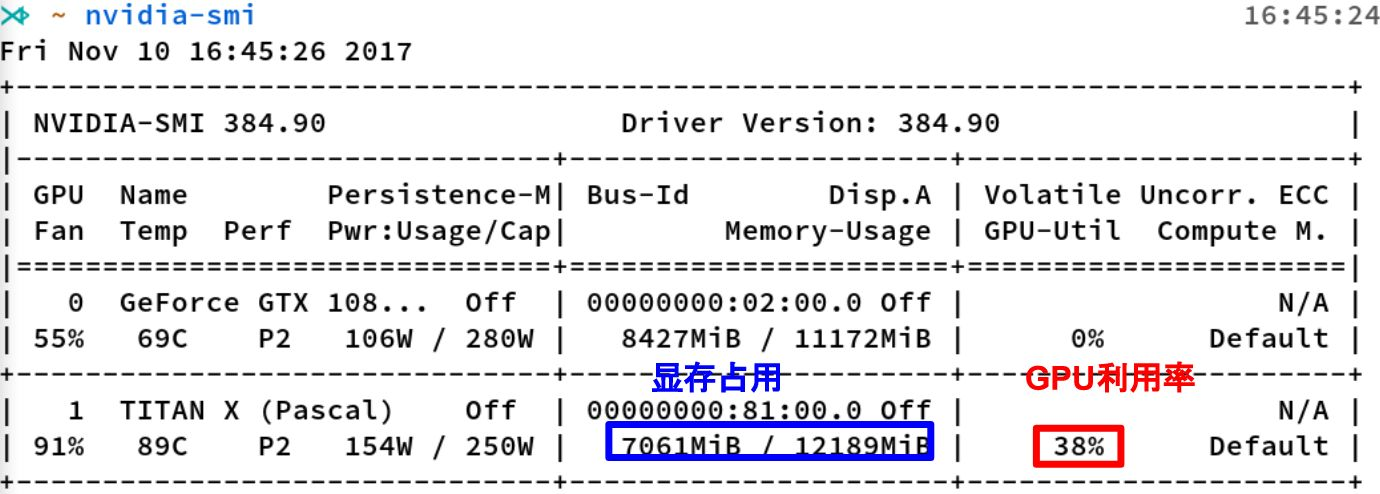• nvidia-smi 是Nvidia显卡命令行管理套件，基于NVML库，旨在管理和监控Nvidia GPU设备
• 显存占用和GPU利用率是两个不一样的东西，显卡是由GPU计算单元和显存等组成的，显存和 GPU的关系有点类似于内存和CPU的关

# 操作系统中，显存与内存的区别？

•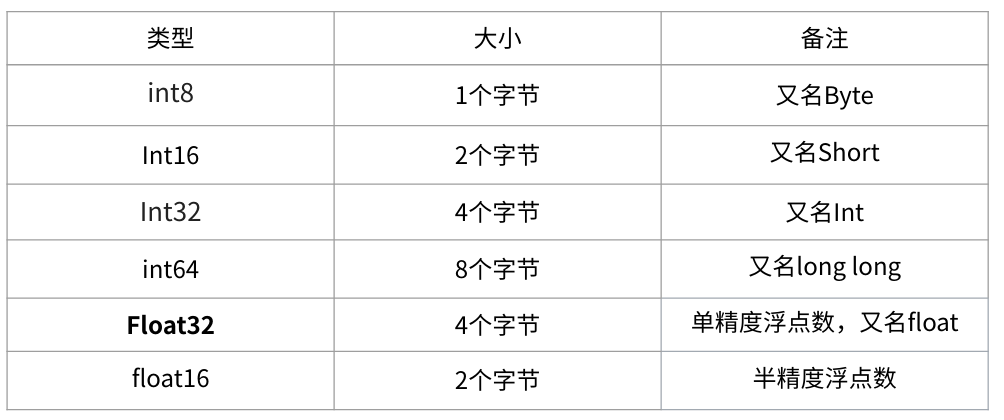• 内存单位K 、 M ， G ， T 是以1024为底，而显存单位KB 、 MB ， GB ， TB 以1000为底。不过一般来说，在 估算显存大小的时候，我们不需要严格的区分这二者
• 在深度学习中会用到各种各样的数值类型，数值类型命名规范一般为 TypeNum ，比如Int64、 Float32、Double64
• 有一个1000x1000的 矩阵，float32，那么占用的显存差不多就是： 1000x1000x4 Byte = 4MB

# 神经网络训练时，哪些部分会占用显存？

• 网络模型占用： 主要指网络中有参数的层，即神经网络中“有参数的层”如何占用显存？
• 模型输入及输出： 训练过程中的中间变量指训练过程需要保存每一层输入输出的中间变量，一般后续反向传导，更新梯度
• 优化器的参数： 除了保存权重之外，还要保存对应的梯度，用于梯度更新，尤其对哪些需要记录历史梯度、自适应学习率的优化器来说
• 框架自身的显存开销

# 神经网络推理时，哪些部分会占用显存？

• “不可控制空间” 指系统分配的空间，如每个进程 CUDA Context 所占用的显存空间，一般在 100-300MB 左右；
• “输入输出” 指用户自行分配的显存空间，如模型输入输出 Tensor 占用的空间，即神经网络中“输入与输出”如何占用显存？
• “模型参数” 指训练的深度学习模型的参数所占用的显存空间，我们需要将模型参数加载到显存中，才能进行计算，即神经网络中“有参数的层”如何占用显存？
• “运行时空间” 是指模型的算子在计算的时候，需要的显存空间

# 神经网络中“输入与输出”如何占用显存？

•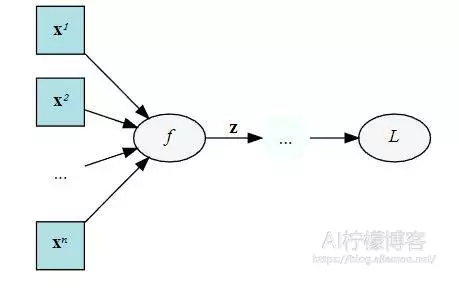• 在训练阶段， 保存模型每一层输出的特征图。（因为反向传播中需要对中间层的特征图求导，所以中间层的输出特征图不会被释放）

$\frac{\partial L}{\partial\mathbf{x}}=\left(\frac{\mathrm{d}\mathbf{z}}{\mathrm{d}\mathbf{x}}\right)^\intercal\frac{\partial L}{\partial\mathbf{z}}\quad\left[\mathbb{R}^{A\times1}=\mathbb{R}^{A\times B}\mathbb{R}^{B\times1}\right]$

• 但是在某些特殊的情况下， 我们可以不要保存输入。比如ReLU，在PyTorch中，使用 nn.ReLU(inplace = True) 能 将激活函数ReLU的输出直接覆盖保存于模型的输入之中，节省不少显存

# 神经网络中“有参数的层”如何占用显存？

•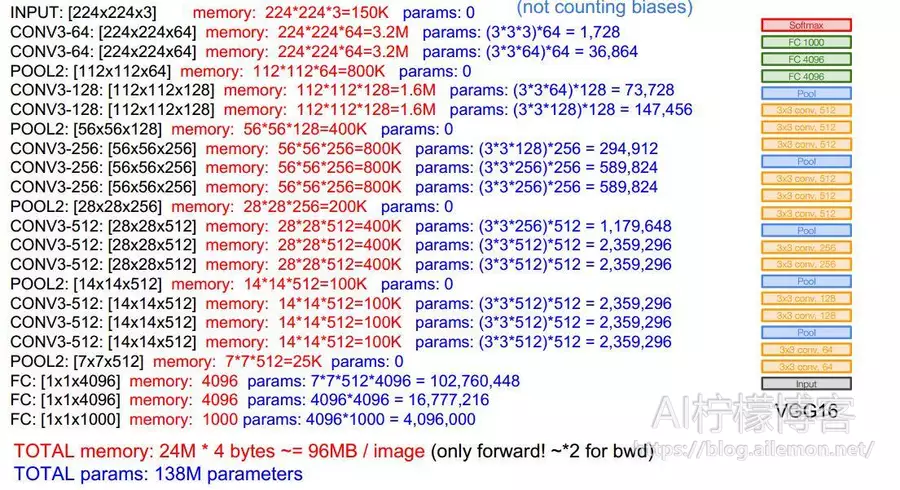• 参数占用显存 = 参数数目×n，其中n为参数数据类型，如float32时，n=4
• 网络模型的占用的显存主要是来自于所有有参数的层，包括：卷积、全连接、BN等；而不 占用显存的有：激活函数、池化层以及Dropout等
• 模型参数的显存占用与batchsize无关，但是在训练阶段与优化器有关

# 神经网络中“梯度及动量”如何占用显存？

•• 在训练阶段， dz/dx这个中间值当然要保留下来以用于计算反向传播更新参数值，所以每个参数都需要存储梯度。所以模型参数 的显存占用，与采用的优化器有关

$\frac{\partial L}{\partial\mathbf{x}}=\left(\frac{\mathrm{d}\mathbf{z}}{\mathrm{d}\mathbf{x}}\right)^\intercal\frac{\partial L}{\partial\mathbf{z}}\quad\left[\mathbb{R}^{A\times1}=\mathbb{R}^{A\times B}\mathbb{R}^{B\times1}\right]$

• 1）如果使用SGD，需要2倍模型参数的显存占用，模型参数+模型参数的梯度

• 2）如果使用SGD+Momentum，需要3倍的模型参数显存占用，模型参数+梯度+动量

# 如何减少显存占用？

• 占用显存较多的部分是卷积层输出的特征图，模型参数的显存占用较少
• (1)减少模型输入尺寸：输入的尺寸不仅仅影响第一层的显存占用，而是每一层的显存占用，参考：神经网络中“输入与输出”如何占用显存？
• (2)减小batchsize：除去模型的显存占用部分，显存占用和batchsize成正比
• (3)尽量使用 Inplace：在PyTorch中，inplce操作指的是改变一个tensor值的时候，不经过复制操作而是直接在原来的内 存上修改它的值，也就是原地操作。
• (4)尽量少产生中间结果： 不需要的中间变量尽可能的都是用一个变量来代替，因为这些变量都是会占用显存的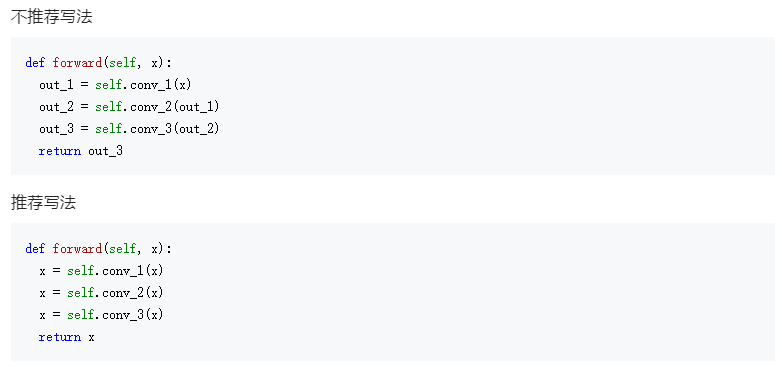• (5)不要使用过大的全连接： 相比于卷积的参数，全连接的参数量可就大多了。因为卷积只是一个局部的连接，而全连接则是 一个全局的连接
• (6)使用checkpoint： PyTorch在0.4版本后推出了一个新功能，可以将一个模型的计算过程分为两半。也就是说，如果 一个模型训练需要占用的显存太大，可以先计算网络的一半，保存后半部分所需要的中奖结果， 再计算后半部分。当然，这样的操作显然是一个牺牲时间换空间的方法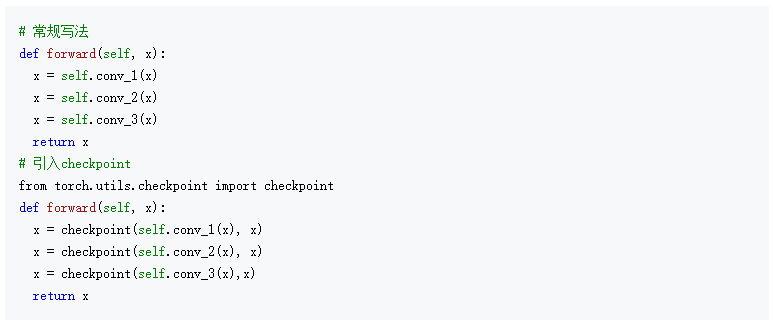• (7)梯度累加： 大多数情况下，其实我们降低显存就是为了获得更大的Batchsize，因此使用gradient accumulation（梯度累加）也可以达到类似的效果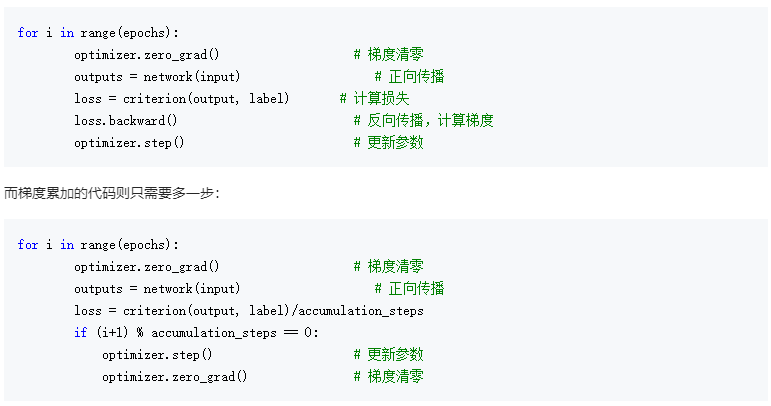• (8)降低计算精度： 在PyTorch1.6之前，降低训练进度普遍使用的都是NVIDIA提供的apex库。而在1.6版本之后， PyTorch推出了AMP（Automatic mixed precision），自动混合精度训练。这套技术并不是简单的 将所有的参数降低精度，而是根据不同向量的不同操作对于误差的敏感程度来决定其使用的是 FP16还是FP32

# 如何减少卷积层的计算量？

• (1) 卷积核的分解（空间可分离卷积(Spatial Separable Convolutions)）： 用两个33的卷积堆叠代替一个77的卷积（达到相同的感受野）
• (2) 深度可分离卷积（深度可分离卷积(depthwise separable convolution)）： 先进行Deepwise卷积操作，再进行Pointwise卷积操作，以空间换取时间，因为显存占用变多(每一步的输出都要保存)

# 为什么神经网络训练时GPU利用率忽高忽低?

• Volfine GPU-Util所说的，当CPU线程数未设置时，此参数反复跳动，0%，20%，70%， 95%，0%。 这休息1-2秒，然后重复。 事实上， GPU 正在等待从 CPU 传输数据。GPU从总线 传输到GPU后，GPU逐渐开始计算，利用率会突然提高。但是，GPU非常强大，基本上可以在 0.5秒内处理数据。然后利用率将再次下降，等待下一批进入。 因此，GPU利用率瓶颈在于内存 带宽和内存介质，以及CPU的性能
]]>
<p>本文分析了深度学习在训练、推理时的显存占用，主要包括：网络参数值、网络参数梯度值、输入+每层输出、优化器参数</p>

# 什么是正则化(regularization)？

• 常见的正则化有L1正则化、 L2正则化、 丢弃正则化(Dropout) ，其中L1正则化倾向稀疏权重(减少参数)、L2正则倾向缩小权重(减小参数)、Dropout倾向减小神经元之间共适应性，相当于在原来模型基础上加入先验信息，也就是说，正则化可以在学习过程中降低模型复杂度和不稳定程度

# 什么是丢弃正则化(Dropout)?

•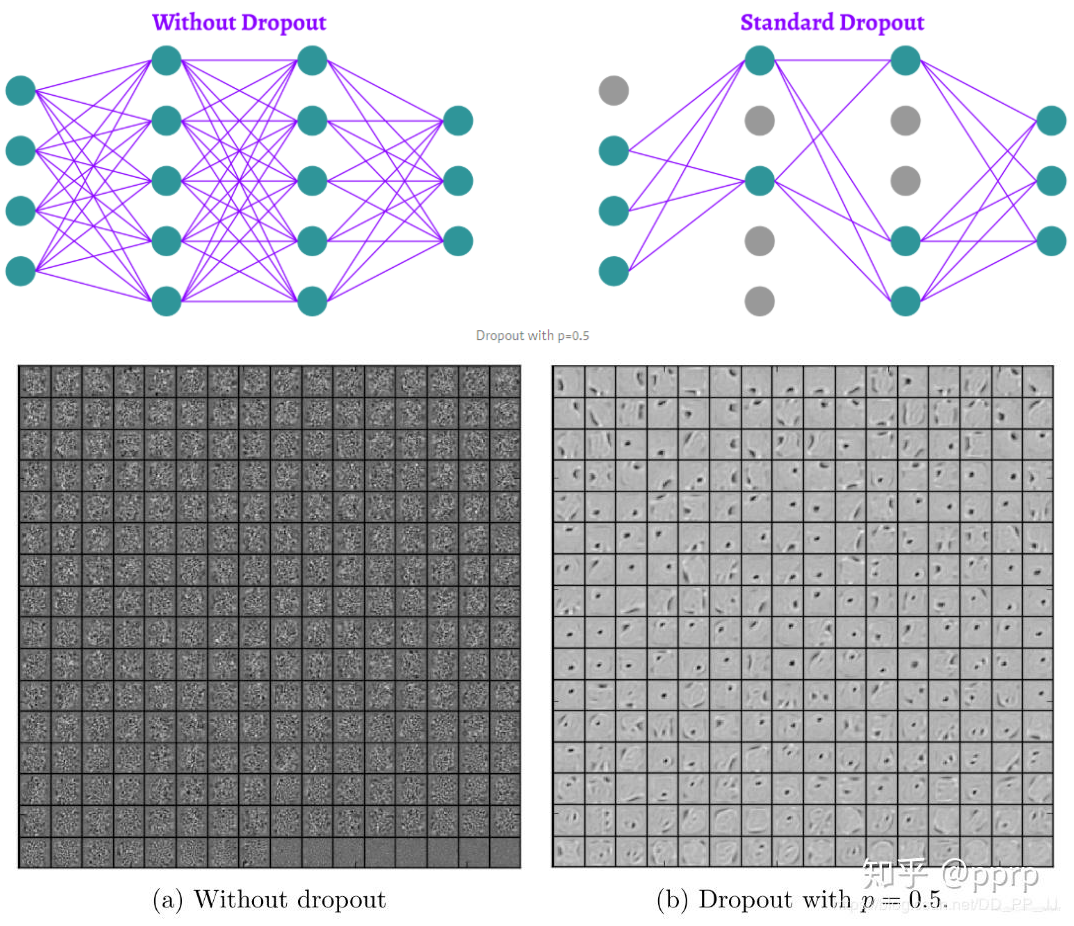• 丢弃正则化 (Dropout) 旨在防止神经网络中单元“共同适应”的方法。换句话说，我们希望神经元独立地从其输入中提取特征，而不是依赖其他神经元来这样做
• 上图是在 MNIST 数据集上进行的 Dropout 实验，可以看到 Dropout 可以破坏隐藏层单元之间的协同适应性，使得在使用 Dropout 后的神经网络提取的特征更加明确，增加了模型的泛化能力

# dropout 的原理?

•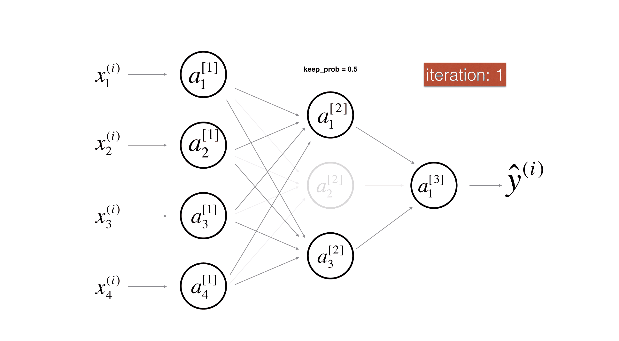• 训练过程：Dropout 的实现是让神经元以超参数 p 的概率停止工作或者激活被置为 0，可以被认为是对完整的神经网络的一些子集进行训练，每次基于输入数据只更新子网络的参数
• 测试过程：不进行随机失活，而是将 Dropout 的参数 p 乘以输出
• 训练时的删除，即指定该该隐单元的输出都为0，如下公式，x 是层输入，W 是权重矩阵，y 是层输出，m 是层 dropout 掩码，遵从概率为 P 的伯努利分布 (bernoulli)，测试时没有 dropout。所有的神经元都是活跃的。为了补偿与训练阶段相比较的额外信息，我们用出现的概率来衡加权权重

$\begin{array}{l}\mathrm{Training~phase}:\\ \quad\quad\mathbf{y}=f(\mathbf{Wx})\circ\mathbf{m},\quad m_i\sim Bernoulli(p)\\ \mathrm{Test~Pbase}:\\ \quad\quad\mathbf{y}=(1-p)f(\mathbf{Wx})\end{array}$

# Dropout如何进行反方向传播？

• 正向传播： 考虑一个输入向量 x , 经丢弃概率为 p 的 dropout 函数变换后得到向量 d, 往前 forward 传播得到误差值 error (标量 e ), 求在训练过程中 e 对 x 的梯度

$\begin{array}{c} x=\left(x_{1}, x_{2}, x_{3}, \cdots, x_{k}\right) \\ d=\operatorname{dopout}(x) \\ e=\text { forward }(d) \end{array}$

• 反向传播

$\begin{array}{c}\nabla e_{(d)}=\left(\frac{\partial e}{\partial d_{1}}, \frac{\partial e}{\partial d_{2}}, \frac{\partial e}{\partial d_{3}}, \cdots, \frac{\partial e}{\partial d_{k}}\right) \\\frac{\partial e}{\partial x_{i}}=\frac{\partial e}{\partial d_{i}} \frac{\partial d_{i}}{\partial x_{i}} \\\frac{\partial d_{i}}{\partial x_{i}}=\left\{\begin{array}{cl}0, & r_{i}

• 梯度向量

$\nabla e_{(x)}=\nabla e_{(d)} \odot\left(m_{1}, m_{2}, m_{3}, \cdots, m_{k}\right)$

# Dropout应用到全连接层？

•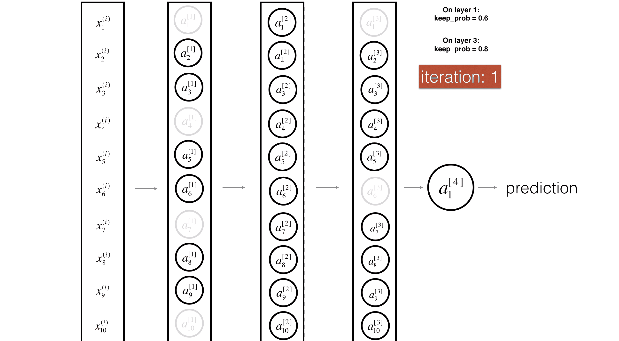# Dropout 应用到卷积层？

•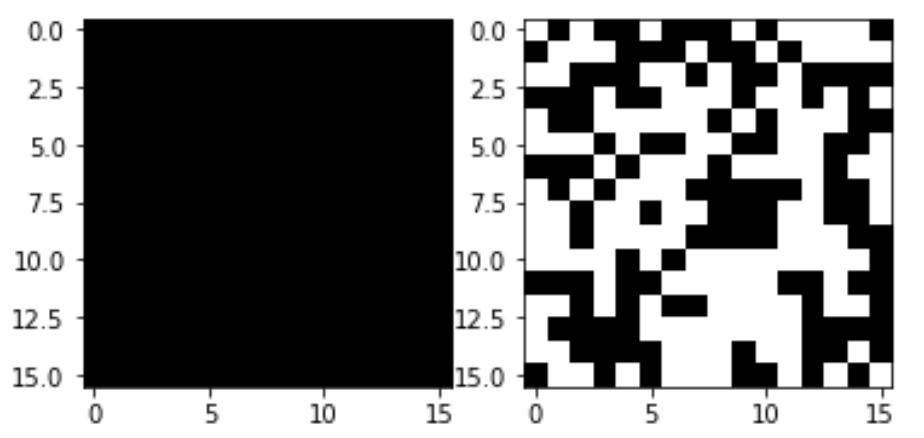• 卷积神经网络 (CNNs)的朴素 dropout定义为特征图或输入图像中随机丢弃像素，并没有显著减少过拟合，主要是因为被丢弃像素的相邻像素具有高度相关性，DropBlock 正则化、Cutout 可应用于卷积神经网络
• 一般很少用普通的Dropout来处理卷积层，这样效果往往不会很理想，原因可能是卷积层的激活是空间上关联的，使用Dropout以后信息仍然能够通过卷积网络传输

## dropout率的选择？

•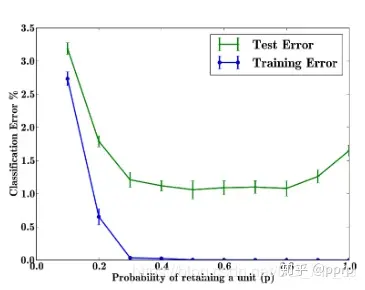• 以上实验是对 MNIST 数据集进行的实验，随着 p 的增加，测试误差先降后升，p 在[0.4, 0.8]之间的时候效果最好，通常 p 默认值会被设为0.5，如果希望在 input 层的添加噪声，可设置为0.8
• 对参数w的训练进行球形限制 (max-normalization)，对 dropout 的训练非常有用， 球形半径c是一个需要调整的参数，可以使用验证集进行参数调优

# 为什么不推荐同时在神经网络中使用 Dropout 和 BN？

•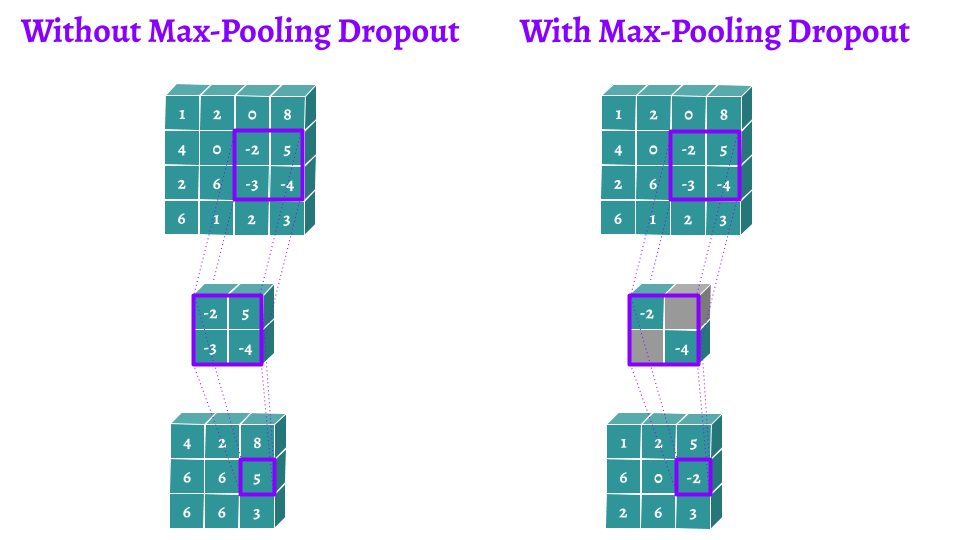• 当丢弃正则化(Dropout)和 批规范化(Batch Normalization,BN) 结合在一起时并不能获得额外的增益。事实上，当一个网络同时使用这两者时，甚至会得到更差的结果
• BN 在某些情况下会削弱 Dropout 的效果。他们揭露了两者之间的不相容性，从而推测 BN 提供了与 Dropout 相似的正则化功能。很多现代模型 如 Resnet，Densenet 等为这一观点添加佐证，它们在只使用 BN 的情况下得到更好的结果

# Dropout 与 Cutout 数据增强的区别？

•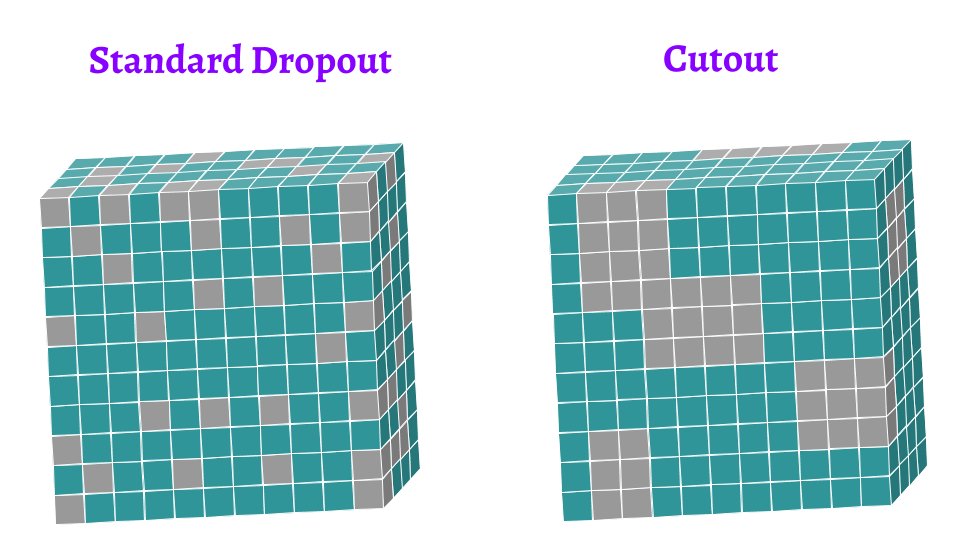• dropout 随机丢弃每个像素，Cutout 可以通过对图像的隐藏区域进行泛化从而限制过拟合

# 什么是DropConnect?

•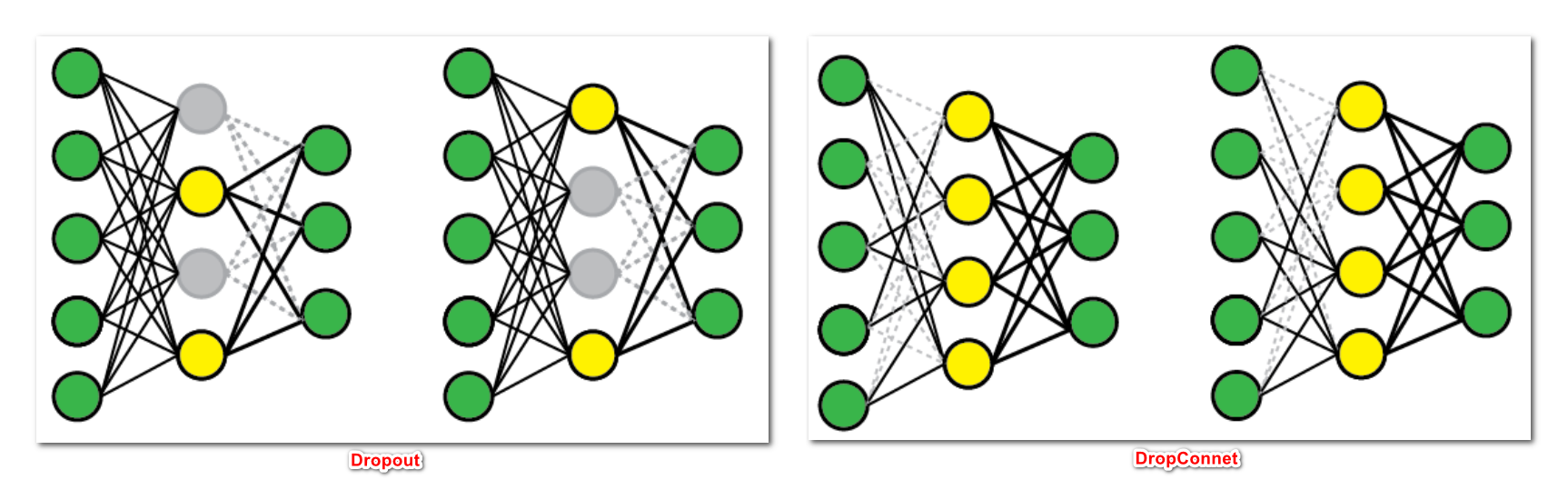• dropout 最早变种之一，它将神经元的权重和偏差以一定概率设置为0，而不是将神经元输出设置为0，其训练时的计算公式为, 其中 M 是掩码矩阵，测试阶段使用与标准 Dropout 方法相同的逻辑。输出乘以出现的概率

$\begin{array}{l}\mathrm{Training Phase}:\\ \quad \quad \mathbf{y}=f((\mathbf{W}\circ\mathbf{M})\mathbf{x}),\quad M_{i,j}\in sim Bernoulli(p)\\ \mathrm{Thing Phase}:\\ \quad \quad \mathbf{y}=(\mathbf{W}\mathbf{x})\circ\hat{\mathbf{m}}(\mathbf{Z})\end{array}$

• DropConnect 是标准 Dropout 的泛化，因为它产生了更多可能的模型，因为几乎权重总是连接多于单元

• 注意： 和 dropout 一样，DropConnect 只能用于全连接的网络层

# 什么是DropPath?

•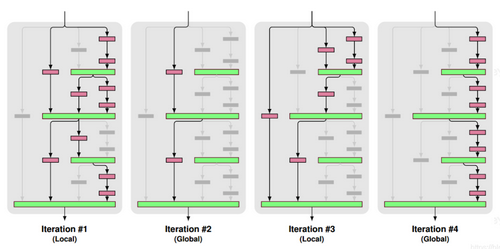• 将深度学习模型中的多分支结构随机失活的一种正则化策略
• 局部丢弃，以一定的概率随机丢弃Join层，但必须保证起码有一条分支是通的
• 全局丢弃，随机选择一条分支

# 什么是Spatial DropOut?

•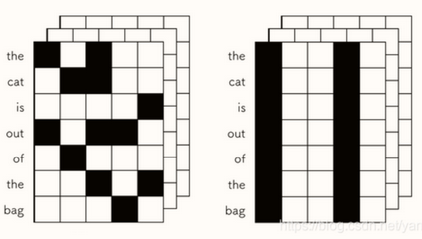• 普通的Dropout会将部分元素失活，而Spatial Dropout则是随机将部分区域失失活，简单理解就是通道随机失活。一般很少用普通的Dropout来处理卷积层，这样效果往往不会很理想，原因可能是卷积层的激活是空间上关联的，使用Dropout以后信息仍然能够通过卷积网络传输。而Spatial Dropout直接随机选取feature map中的channel进行dropout，可以让channel之间减少互相的依赖关系
• Spatial Dropout 常用于 NLP 中（Embedding 中）

# 什么是 Cutout 数据增强？

•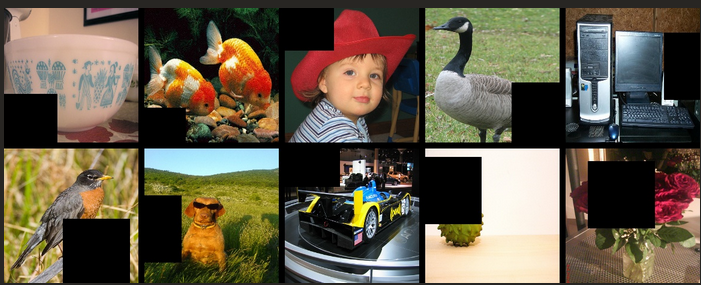• Cutout 的出发点和 Random Erasing 一样，也是模拟遮挡，目的是提高泛化能力，实际上比 Random Erasing 简单，随机选择一个固定大小的正方形区域，然后采用全 0 填充
• Cutout 区域的大小比形状重要，所以 Cutout 只要是正方形就行。具体操作是利用固定大小的矩形对图像进行遮挡，在矩形范围内，所有的值都被设置为 0，或者其他纯色值，而且擦除矩形区域存在一定概率不完全在原图像中（文中设置为 50%）
• Cutout 可以理解为 Dropout 的一种扩展操作，不同的是 Dropout 是对图像经过网络后生成的特征进行遮挡，而 Cutout 是直接对输入的图像进行遮挡，相对于 Dropout，Cutout 对噪声的鲁棒性更好

# 什么是 DropBlock？

•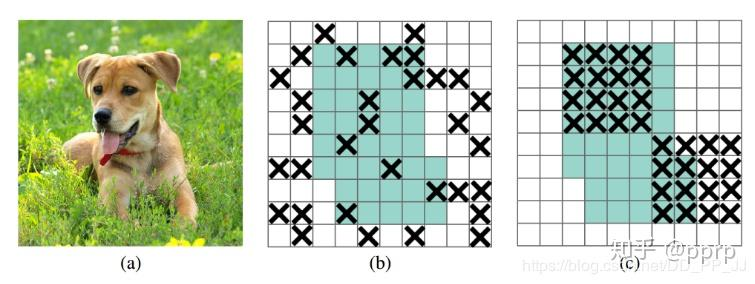• Dropout 对卷积层的效果没那么好（图 (b)）。文章认为是由于每个 feature map 中的点都对应一个感受野范围，仅仅对单个像素位置进行 Dropout 并不能降低 feature map 学习的特征范围，网络依然可以通过失活位置相邻元素学习对应的语义信息
• DropBlock 有三个重要的参数：1）block size 控制 block 的大小；2）γ 控制有多少个 channel 要进行 DropBlock；3）keep prob 类别 Dropout 中的 p，以一定的概率失活

# Dropout、DropBlock、SpatialDrop、DropPath的联系？

•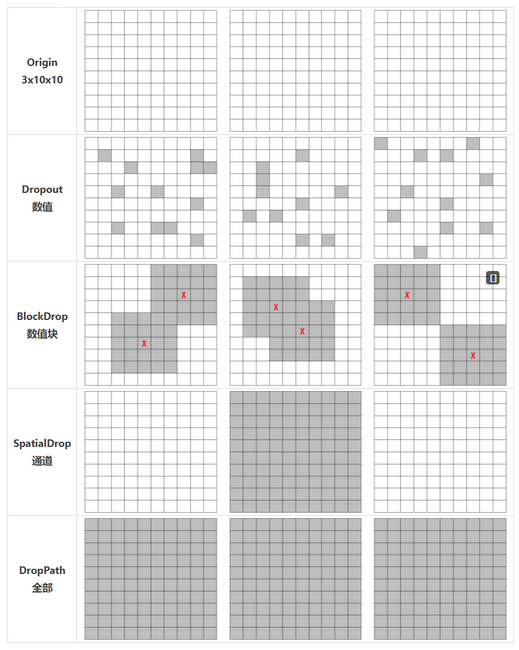• DropOut：对NCHW 中所有的特征进行随机失活, 以像素为单位，用在全连接层较多，在卷积层基本不用（BN 代替）
• DropBlock：随机对 $C[HW]_{part}$ 进行失活，用在卷积层，效果很好，比如 YOLOv4
• Spatial Dropout：随机将 CHW 的特征进行随机失活，以 channel 为单位，常用在 NLP 的 Embedding 中
• DropPath：随机跳过一个 Res Block, 单位更大，用在 Block 支路比较多的模型中
• DropConnect：让Weight随机失活，而不是unit

]]>
<p>在训练神经网络时，以使神经某些神经元失活的方式增强模型的泛化能力，还要掌握Dropout在训练时和推理时的差异</p>

# 什么是优化器？

•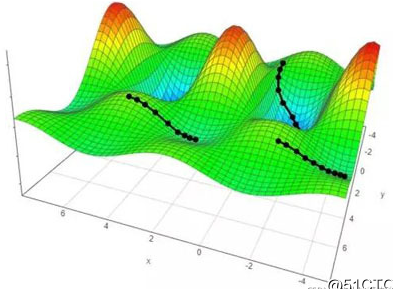• 优化器在深度学习反向传播过程中，指引损失函数（目标函数）的各个参数往正确的方向进行适合的更新，使得更新后的各个参数让损失函数（目标函数）值不断逼近全局最小
• 如何求最小值呢？对于凸函数可以直接求导可以找到最值，但是神经网络的优化本质上是一个非凸问题，而简单的基于梯度的优化器在实践中似乎总是能够解决这类问题

# 深度学习中的优化挑战有哪些？

• 病态情况： 在优化凸函数的时候，会遇到Hessian矩阵(黑塞矩阵) 病态的情况。病态情况一般被认为广泛存在于神经网络的训练过程中，体现在随机梯度下降会“卡”在某些特殊的情况，此时即使很小的更新步长也会增加代价函数
• 局部最小值： 当优化问题的数值解接近局部最优值时，随着目标函数解的梯度接近或变为零，通过最终迭代获得的数值解可能仅使目标函数局部最优，而不是全局最优。只有一定程度的噪声可能会使参数超出局部最小值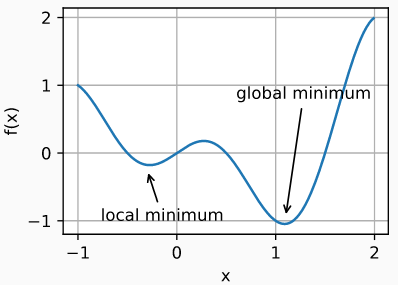• 鞍点 ： 指函数的所有梯度都消失但既不是全局最小值也不是局部最小值的任何位置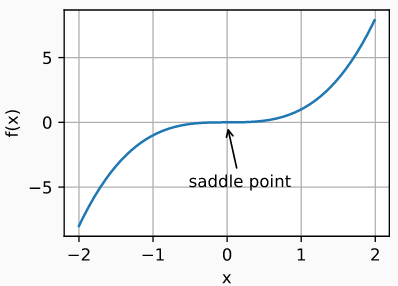# 深度学习有哪些优化函数？

•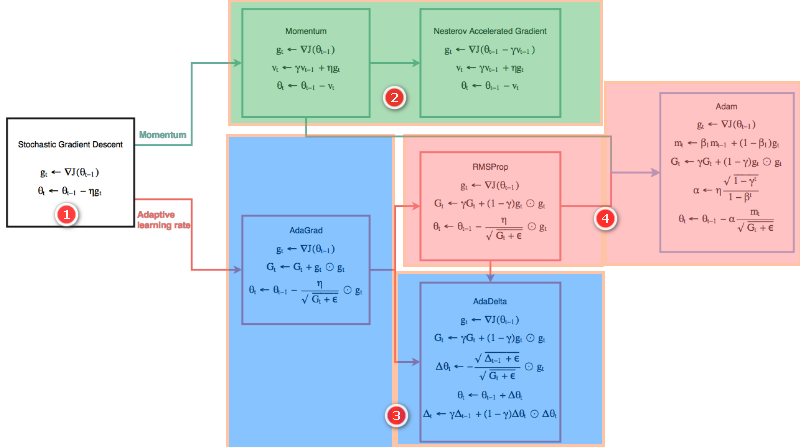• 2）加强版梯度下降法 (带一阶动量的梯度)：带动量 (Momentum)项、Nesterov 动量项
• 基于动量的优化器可以利用历史梯度参与参数更新；基于自适应学习率通过约束学习率达到快速收敛的目的

# 如何选择优化算法？

•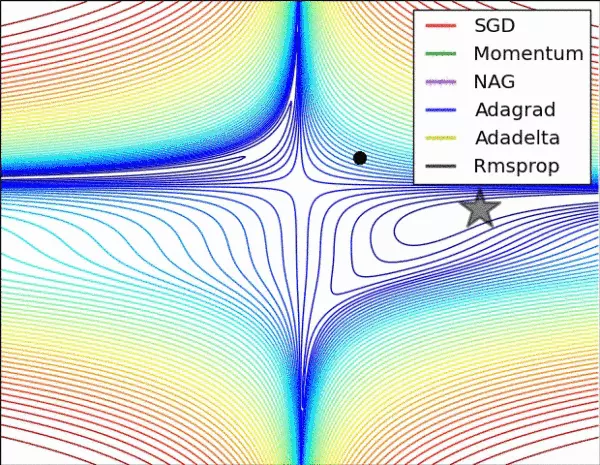• 如果你的数据偏小而且能够适应一次迭代，那么就可以选择诸如 LBFGS这样的二阶技术。这是因为，二阶技术虽然速度非常快并且非常准确，但是只适用于数据偏小的情况。

# Pytorch如何求梯度？

• 即求取神经网络中输出相对于输入参数的变化率，以便确定神经网络中参数的调整方向及调整尺度， 并最终达到减少网络输出与真实结果的差异
• 求取 out=mean ((x+2) * (x+2) * 3)的梯度，假设 $z=(x+2)*(x+2)*3$ ，则导数为：$z=3*(x+2)/2$ ，当 x=1时，导数值为4.5

• 采用整个训练集的数据来计算梯度，计算起来非常慢，遇到很大量的数据集也会非常棘手，而且不能投入新数据实时更新模型

•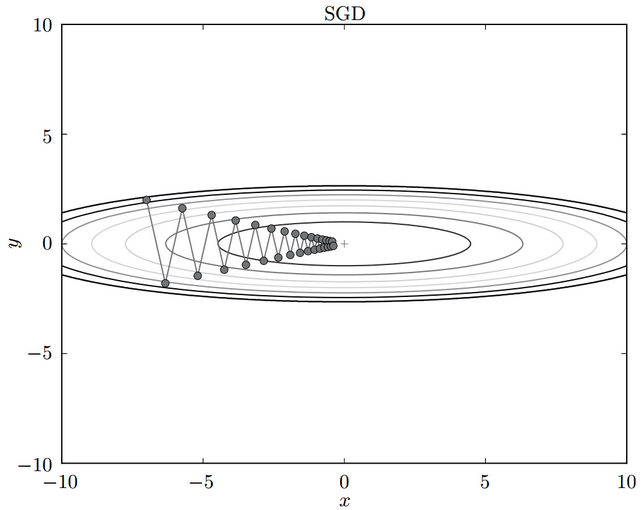• 不像批量梯度下降(Batch Gradient Descent, BGD)一次用所有数据计算梯度，SGD每次更新时对每个样本进行梯度更新，对于很大的数据集来说，可能会有相似的样本，这样 BGD 在计算梯度时会出现冗余，而 SGD 一次只进行一次更新，就没有冗余，而且比较快，并且可以新增样本

$W \leftarrow W-\eta \frac{\partial L}{\partial W}$

• 可以看到BGD和SGD是两个极端，SGD由于每次参数更新仅仅需要计算一个样本的梯度，训练速度很快，即使在样本量很大的情况下，可能只需要其中一部分样本就能迭代到最优解，由于每次迭代并不是都向着整体最优化方向，导致梯度下降的波动非常大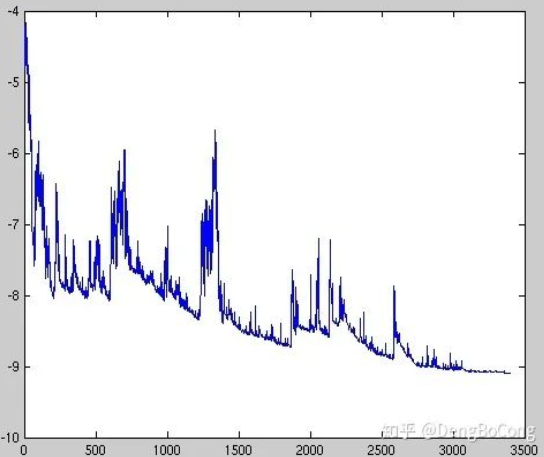• 虽然看起来SGD波动非常大，会走很多弯路，但是对梯度的要求很低（计算梯度快），而且对于引入噪声，大量的理论和实践工作证明，只要噪声不是特别大，SGD都能很好地收敛

# 什么是小批量梯度下降 (Mini-Batch Gradient Descent, MBGD)?

• 小批量梯度下降法就是结合批量梯度下降(Batch Gradient Descent, BGD)和随机梯度下降(stochastic gradient descent, SGD) 的折中，对于含有$n$个训练样本的数据集，每次参数更新，选择一个大小为
• Mini-batch gradient descent 不能保证很好的收敛性，学习速率 (learning rate)如果选择的太小，收敛速度会很慢，如果太大，损失函数就会在极小值处不停地震荡甚至偏离
• 对于非凸函数，还要避免陷于局部极小值处，或者鞍点处，因为鞍点所有维度的梯度都接近于0，SGD很容易被困在这里
• Batch size的选择参考：如何选择合理的batch size?

# 什么是在线梯度下降(Online GD）?

• Online GD在互联网领域用的较多，比如搜索广告的点击率（CTR）预估模型，网民的点击行为会随着时间改变。用普通的BGD算法（每天更新一次）一方面耗时较长（需要对所有历史数据重新训练）；另一方面，无法及时反馈用户的点击行为迁移。而Online GD算法可以实时的依据网民的点击行为进行迁移

# 为什么选择Mini-Batch进行梯度下降，而不是1个或全部样本？

• 小批量梯度下降(Mini-Batch Gradient Descent, MBGD)用部分样本来近似全部样本，梯度相对于batch size为1更为准确，同时相比与使用全部样本，计算量减小，计算速度和收敛速度都会得到提升
• 为什么小批量的梯度下降更快，详细查看：为什么10000样本训练1次会比100样本训练100次收敛慢呢？

# 随机梯度下降（SGD）、批量梯度下降（BGD）、小批量梯度下降（Mini-batch GD）、和Online GD的区别?

•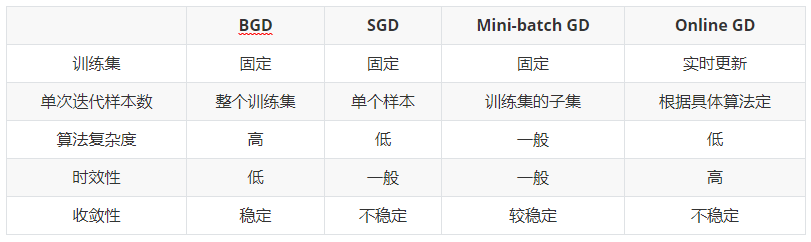# SGD与自适应算法的区别？

• 随机梯度下降(stochastic gradient descent, SGD) 在更新参数时对各个维度上梯度的放缩是一致的，并且在训练数据分布极不均很时训练效果很差，所以SGD前期收敛慢

# 如何理解深度学习中带动量(Momentum)的优化算法？

•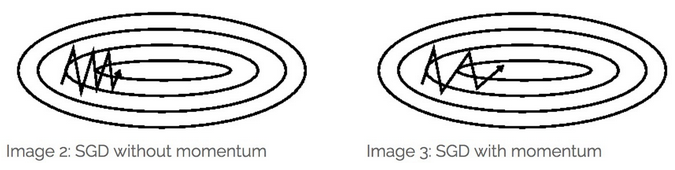• 随机梯度下降(stochastic gradient descent, SGD) 和小批量梯度下降(Mini-Batch Gradient Descent, MBGD)算法都存在样本选择的随机性，因此含有较多的噪声，而momentum技术能解决上述噪声问题，借助类似指数加权平均(exponentially weighted averges) ，利用历史梯度参与参数更新。权重更新变为

$\begin{array}{c} v_{t+1} \leftarrow \alpha v_{t}-\eta \frac{\partial L}{\partial W_{t}} \\\\ W_{t+1} \leftarrow W_{t}+v_{t+1} \end{array}$

• 其中$v_{t+1}$ 是动量项，取代之前的梯度$\frac{\partial L}{\partial W_{t}}$，它是上一时刻的动量项本次梯度值的加权平均值，按照时间展开，可知$V_{t+1}$会按指数级累积以前的动量；$\alpha$ 是学习率，$\mu$ 是动量系数

• 加入的这一项，可以使得梯度方向不变的维度上速度变快，梯度方向有所改变的维度上的更新速度变慢，这样就可以加快收敛并减小震荡， 一般 γ 取值 0.9 左右

• 形象地说，动量法就像我们从山上推下一个球，在滚落过程中累积动量，速度越来越快，直到达到终极速度。在梯度更新过程中，对在梯度点处具有相同方向的维度，增大其动量项，对于在梯度点处改变方向的维度，减小其动量项

•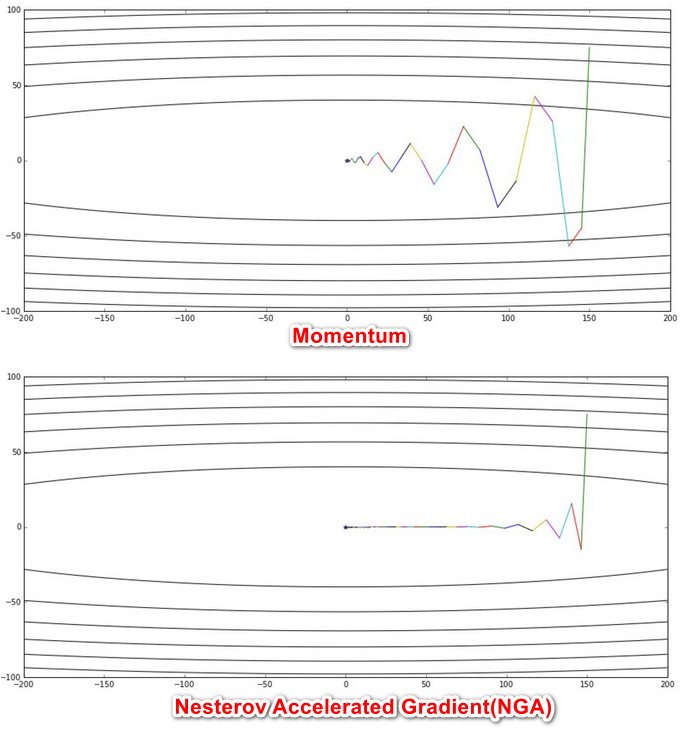• NAG全称Nesterov Accelerated Gradient，是在随机梯度下降 (SGD) 、带动量(Momentum)项的基础上的进一步改进，我们知道在时刻t的主要下降方向是由累积动量决定的，自己的梯度方向说了也不算，那与其看当前梯度方向，不如先看看如果跟着累积动量走了一步，那个时候再怎么走。因此，NAG不计算当前位置的梯度方向，而是计算如果按照累积动量走了一步，给动量项一个预知的能力，即梯度“多走一步”，可能跳出局部最优解

$\begin{array}{c} v_{t+1} \leftarrow \alpha v_{t}-\eta \frac{\partial L\left(W_{t}+\alpha v_{t}\right)}{\partial W_{t}} \\\\ W_{t+1} \leftarrow W_{t}+v_{t+1} \end{array}$

• 我们利用动量项$αv_t$更新参数$w_t$，通过计算($W_t+αv_t$)得到参数未来位置的一个近似值，计算关于参数未来的近似位置的梯度，而不是关于当前参数Wt的梯度，这样NAG算法可以高效地求解

• 相对于Momentum的改进在于，NAG算法相对于Momentum多了一个本次梯度相对上次梯度的变化量，这个变化量本质上是对目标函数二阶导的近似。由于利用了二阶导的信息，NAG算法才会比Momentum具有更快的收敛速度

• 形象地说，当球滚落的时候，一般是盲目地沿着某个斜率方向，结果并不一定能令人满意。于是我们希望有一个较为“智能”的球，能够自己判断下落的方向，这样在途中遇到斜率上升的时候能够知道减速

$h \leftarrow h+\frac{\partial L}{\partial W} \cdot \frac{\partial L}{\partial W}$

• 在更新参数时，通过乘以$\frac {1} {\sqrt{h}}$ ，就可以调整学习的尺度，前期$g_t$较小的时候，$\frac {1} {\sqrt{h}}$较大，能够放大梯度；后期$g_t$较大的时候，$\frac {1} {\sqrt{h}}$较小，能够约束梯度，适合处理稀疏梯度

$W \leftarrow W-\frac{\eta}{\sqrt{h}} \cdot \frac{\partial L}{\partial W}$

$\begin{array}{c} h_{t} \leftarrow \alpha h_{t-1}+(1-\alpha) \frac{\partial L}{\partial W_{t}} \cdot \frac{\partial L}{\partial W_{t}} \\\\ \Delta x_{t}=\frac{\sqrt{\sum_{k=1}^{t-1} \Delta x_{k}}}{\sqrt{h_{t}}} \frac{\partial L}{\partial W} \\\\ W \leftarrow W-\Delta x_{t} \end{array}$

# 什么是RMSProp自适应优化算法？

$\begin{array}{c} h \leftarrow \alpha h+(1-\alpha) \frac{\partial L}{\partial W} \cdot \frac{\partial L}{\partial W} \\\\ W \leftarrow W- \frac{\eta}{\sqrt{h}} \frac{\partial L}{\partial W} \end{array}$

$m_{t} \leftarrow \beta_{1} m_{t-1}+\left(1-\beta_{1}\right) \frac{\partial L}{\partial W} \\\\ v_{t} \leftarrow \beta_{2} v_{t-1}+\left(1-\beta_{2}\right) \frac{\partial L}{\partial W} \cdot \frac{\partial L}{\partial W} \\\\$

• 对一阶动量与二阶动量进行校正，并按下公式更新参数

$\begin{array}{c} \hat{m}_{t}=\frac{m_{t}}{\left(1-\beta_{1}\right)} \\\\ \hat{v}_{t}=\frac{v_{t}}{\left(1-\beta_{2}\right)} \\\\ W_{t}=W_{t-1}- \frac{\alpha}{\sqrt{\hat{v}_{t}}} \hat{m}_{t} \end{array}$

• 可以看出，Adam会设置3个超参数，即学习率$\alpha$ ，一次momentum系数$\beta_1$，二次momentum系数$\beta_2$

• AMSGrad是一个随机梯度下降优化方法，它试图解决基于Adam的优化器的收敛问题。AMSGrad使用最大化过去平方梯度$v_t$来更新参数，而不是使用指数平均，这样就降低了指数衰减平均，造成重要历史信息快速丢失的影响

$\begin{array}{c} m_{t} =\beta_{1} m_{t-1}+\left(1-\beta_{1}\right) g_{t} \\ \\ v_{t} =\beta_{2} v_{t-1}+\left(1-\beta_{2}\right) g_{t}^{2}\\ \\ \hat{v}_{t}=\max \left(\hat{v}_{t-1}, v_{t}\right)\\ \\ \theta_{t+1}=\theta_{t}-\frac{\eta}{\sqrt{\hat{v}_{t}}+\epsilon} m_{t} \end{array}$

• $g_t$ 是当前参数的梯度，$β_1$为一阶矩估计的指数衰减率，$β_2$是二阶矩估计的指数衰减率，前者控制一阶矩估计，后者控制二阶矩估计，从上面的公式可以看出，参数更新公式与Adam没有啥区别，但是求$\hat{v}_{t}$有区别

$\begin{array}{c} m_{t} =\beta_{1} m_{t-1}+\left(1-\beta_{1}\right) g_{t} \\ \\ v_{t} =\beta_{2} v_{t-1}+\left(1-\beta_{2}\right) g_{t}^{2}\\ \\ V_{t}=\operatorname{diag}\left(v_{t}\right) \\ \\ \left.\hat{\eta}_{t}=\operatorname{Clip}\left(\alpha / \sqrt{(} V_{t}\right), \eta_{l}(t), \eta_{u}(t)\right) \\ \\ \eta_{t}=\hat{\eta}_{t} / \sqrt{t}\\\\ \theta_{t+1}=\theta_{t}-\eta_{t} \odot m_{t} \end{array}$

• 把学习率限定在$[\eta_{l}, \eta_{u}]$之间，其中$\eta_{l}$是一个非递减函数，从0逐渐的收敛到α，而$\eta_{u}$是一个非递增函数，从∞逐渐收敛到α , 在这种设置下，AdaBound在最开始表现的像Adam，因为最开始学习率的边界对更新公式影响很小，渐渐的表现的像SGD+momentum，因为学习率逐渐被限制住了

$\begin{array}{l} m_{t}=\beta_{1} m_{t-1}+\left(1-\beta_{1}\right) \nabla L\left(\theta_{t-1}\right) \\ v_{t}=\beta_{2} v_{t-1}+\left(1-\beta_{2}\right)\left(\nabla L\left(\theta_{t-1}\right)\right)^{2} \\ \theta_{t}=\theta_{t-1}-\eta\left(\frac{1}{\sqrt{\hat{v}_{t}}+\epsilon} \hat{m}_{t}-\gamma \theta_{t-1}\right) \end{array}$

•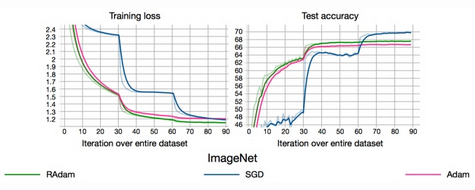• Slow Weights： 在每轮内循环结束后，根据本轮的k次权重，计算等到Slow Weights；这里采用的是指数移动平均（exponential moving average, EMA）算法来计算，最终模型使用的参数也是慢更新（Slow Weights）那一套，因此快更新（Fast Weights）相当于做了一系列实验，然后慢更新再根据实验结果选一个比较好的方向，这有点类似 Nesterov Momentum 的思想

• 根据链式法则，如果每一层神经元对上一层的输出的梯度乘上权重结果都小于1的话，那么即使这个结果是0.99，在经过足够多层传播之后，误差对输入层的梯度会趋于0
• 当梯度消失发生时，接近于输出层的隐藏层由于其梯度相对正常，所以权值更新时也就相对正常，但是当越靠近输入层时，由于梯度消失现象，会导致靠近输入层的隐藏层权值更新缓慢或者更新停滞。这就导致在训练时，只等价于后面几层的浅层网络的学习

• 根据链式法则，如果每一层神经元对上一层的输出的梯度乘上权重结果都大于1的话，那么即使这个结果是1.01，在经过足够多层传播之后，误差对输入层的梯度会趋于无穷

# 为什么会出现梯度消失会梯度爆炸？

• 深层网络导致浅层网络可能出现梯度消失或梯度爆炸
• 饱和激活函数导致梯度传播趋向0，可能出现梯度消失
• 梯度消失和梯度爆炸本质上是一样的，都是因为网络层数太深而引发的梯度反向传播中的连乘效应

# 如何解决梯度消失与梯度爆炸？

• 使用Relu 、Leaky-ReLU 、elu 等不饱和激活函数
• 使用 批规范化(Batch Normalization,BN)
• 使用 ResNet结构
• 逐层训练+ finetunning ： 每次训练一层隐藏层节点，将上一层隐藏层的输出作为输入，而本层隐节点的输出作为下一层隐节点的输入，这就是逐层预训练
• 梯度正则化(regularization)： 检查网络权重的大小，并惩罚产生较大权重值的损失函数

• 在应用梯度值之前先设置其上限。梯度裁剪有助于确保数值稳定性以及防止梯度爆炸

# 简单堆叠的神经网络，梯度在各层神经网络的更新特点？

•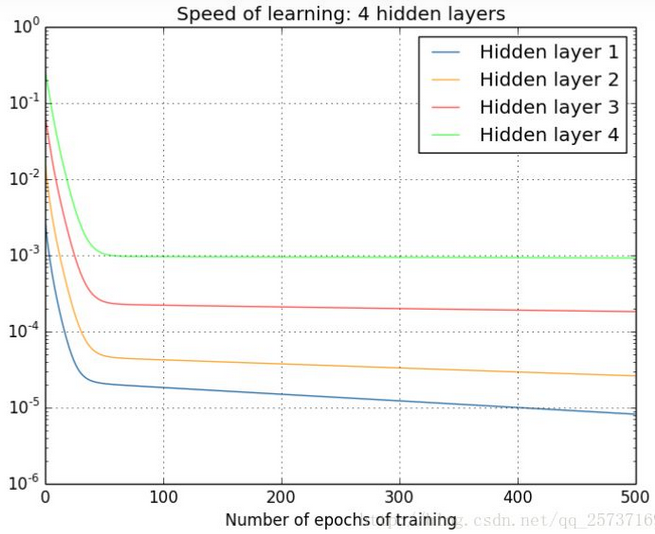• 从深层网络角度来讲，不同的层学习的速度差异很大，表现为网络中靠近输出的层学习的情况很好，靠近输入的层学习的很慢，有时甚至训练了很久，前几层的权值和刚开始随机初始化的值差不多

# 深度学习为什么不使用二阶优化算法？

• 速度慢： 计算量大，训练非常慢
• 稳定性： 二阶方法能更快求高精度的解，同样对数据本身要的精度也会相应的变高，这就会导致稳定性上的问题
• 二阶方法能够更快地求得更高精度的解，这在浅层模型是有益的，而在神经网络这类深层模型中对参数的精度要求不高，甚至不高的精度对模型还有益处，能够提高模型的泛化能力
]]>

# 什么是损失函数？

•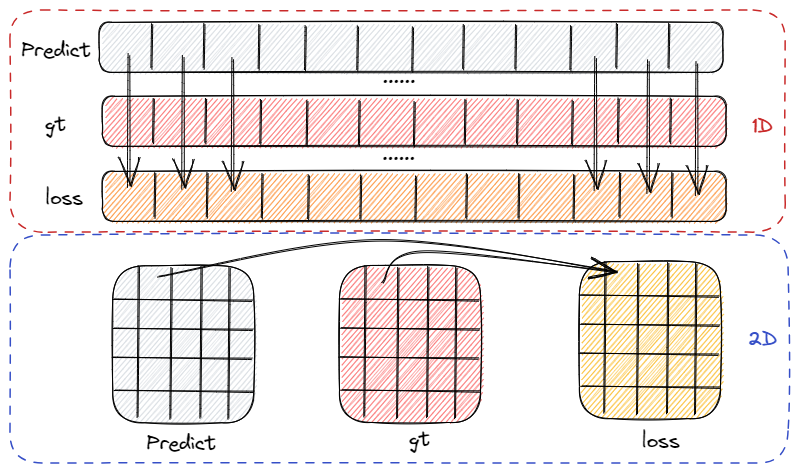• 损失函数是用来度量模型预测值和真实值间的偏差，偏差越小，模型越好，所以我们需要最小化损失函数
• 神经网络的学习过程就是不断减小模型损失的过程，这个过程设计 2 个步骤：1）计算损失函数；2）通过优化函数修改参数以减小损失值
• 计算损失值的过程是针对每个预测点位计算损失，如图是 1D、2D 场景下的损失计算示意图，每个点位都有对应损失。计算过程可以是只有对应位置参加，如 MSE，交叉熵，也可以是全局点位参加，如 Dice loss

# 损失函数和目标函数的区别？

• 损失函数(代价函数)： 损失函数越小，就代表模型拟合的越好
• 目标函数： 把要最大化或者最小化的函数（模型迭代时的迭代方向，往目标函数最小化的方向迭代）称为目标函数，有正则化 (regularization) 的必定是目标函数

# 什么是绝对值损失(L1Loss)？

• 等价于 L1损失，相当于在做中值回归，相比做均值回归的平方损失函数，绝对损失函数对异常点更鲁棒

$L(Y, f(x)) = |Y-f(x)|$

# 什么是平方损失(MSELoss/L2Loss)?

• 等价于 L2损失，是光滑函数，能够使用梯度下降法优化。然而当预测值距离 gt 是只越远时，平方损失函数的惩罚力度越大，因此对异常点比较敏感

$L(Y, f(x)) =\frac {1} {2} \sum_N{(Y-f(x))}^2$

# 平方损失 (MSELoss/L2Loss) 的梯度分析？

•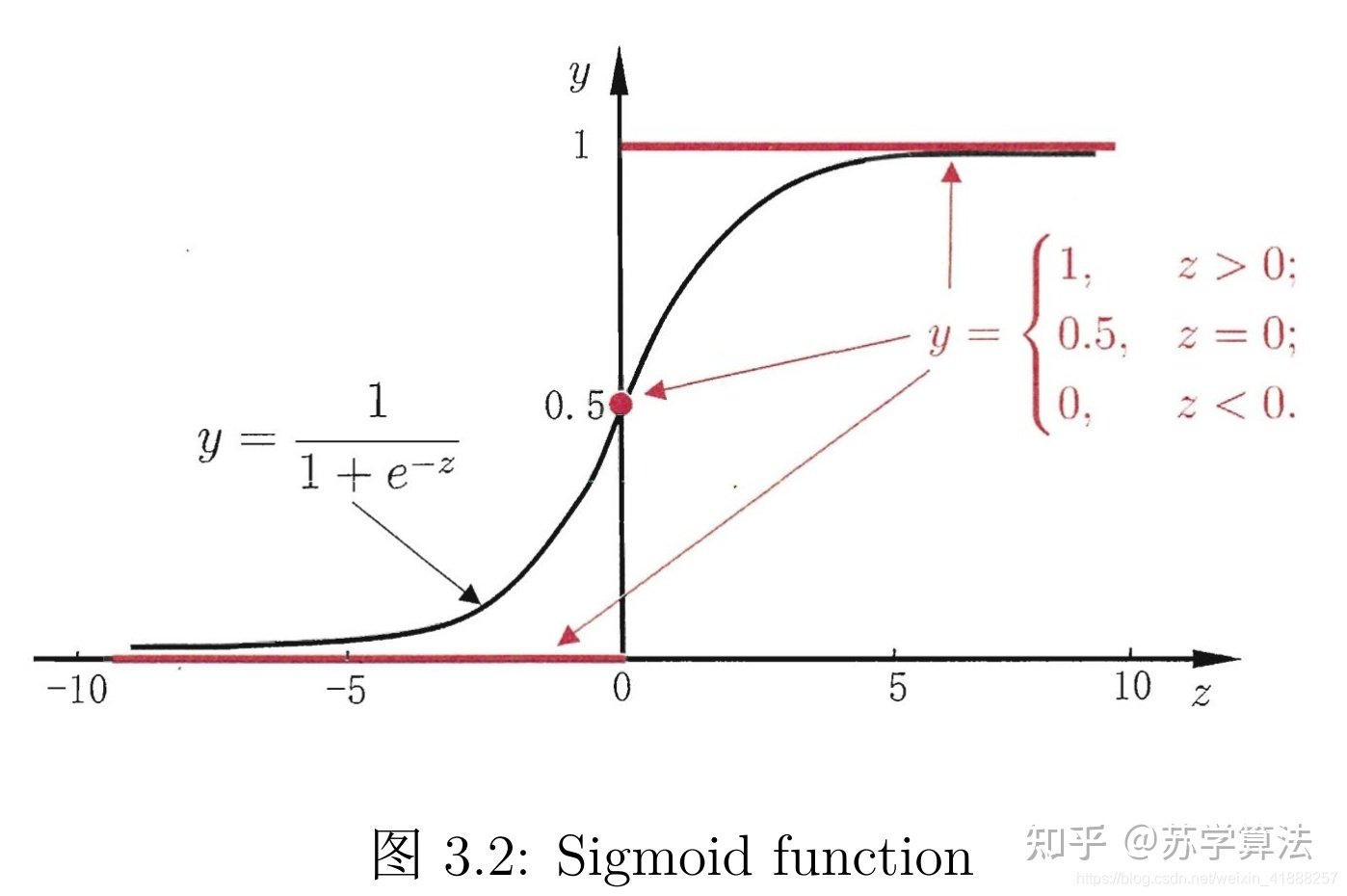• 以下公式 y 是期望输出，Y 是实际输出，z=wx+b，Y=sigmoid (z)，已知 Y 关于 z 的梯度是 Y (1-Y)，则 MSE 梯度为

\begin{aligned}\frac{\partial C}{\partial w} =\frac{\partial C}{\partial z}\frac{\partial z}{\partial w}=(Y-y)x\sigma'(z)=(Y-y)Y(1-Y)x\approx Y\sigma'(z)\\ \frac{\partial C}{\partial b} =(Y-y)\sigma'(z)=(Y-y)Y(1-Y)\approx Y\sigma'(z)\end{aligned}

• 网络更新慢：因为 sigmoid 函数的性质，如图的两端，几近于平坦，导致\sigma’ (z)在 z 取大部分值时会很小，这样会使得 w 和 b 更新非常慢

• 无法区分小梯度：y=1, Y=0 、y=1, Y=1、y=0, Y=1、y=0, Y=0 的梯度一样，都是 0，当梯度很小的时候，MSE无法知道是离目标很远还是已经在目标附近了。（离目标很近和离目标很远，其梯度都很小）

# 什么是平滑绝对值损失(SmoothL1Loss)?

•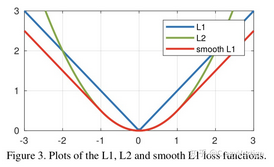• 又称Huber Loss，在Fast RCNN提出的损失函数，是在结合绝对值损失(L1Loss) 、平方损失(MSELoss/L2Loss)的基础上提出的

$L(Y,f(x))= \begin{cases}0.5 [Y-f(x)]^{2} & |Y-f(x)|<1 \\ |Y-f(x)|-0.5 & \text { otherwise }\end{cases}$

• 一阶导数：

$\frac{d s m o o t h L 1_{1}(x)}{x}=\left\{\begin{array}{rr} f(x) & \text { if }|f(x)|<1 \\ \pm 1 & \text { otherswise } \end{array}\right.$

• L1损失函数为常数，在训练后期，Y与f(x)很小时，如果学习速率 (learning rate) 不变，损失函数会在稳定值附近波动，很难收敛到更高的精度；L2损失函数对x的导数在x值很大时，其导数也非常大，在训练初期不稳定。 Smooth L1 Loss完美避开了L1、L2损失的缺点

# 什么是交叉熵损失 (CE Loss) ?

•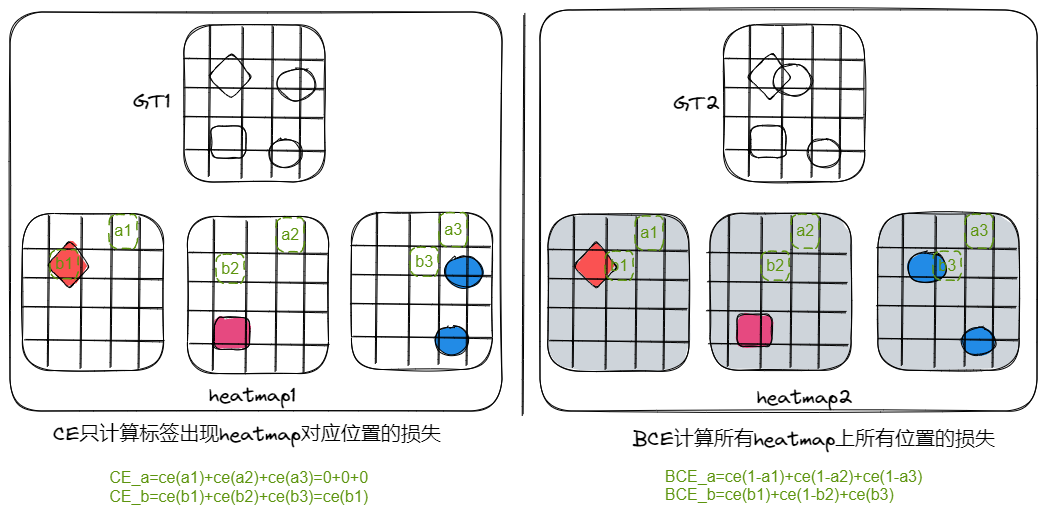• 交叉熵(CrossEntropy) 就是交差熵损失，该值表示实际输出（概率）与期望输出（概率）分布的距离，也就是交叉熵的值越小，两个概率分布就越接近

• 二分类 (BCE)$y_i$ 表示样本 i 的标签，正类为1，负类为0；$p_i$ 为样本 i 为正类的概率

$L=\frac{1}{N} \sum_{i} L_{i}=\frac{1}{N} \sum_{i}-\left[y_{i} \cdot \log \left(p_{i}\right)+\left(1-y_{i}\right) \cdot \log \left(1-p_{i}\right)\right]$

• 多分类 (CE)$M$ 为类别数量；$y_i$ 表示样本 i 的标签， $p_{ic}$ 为样本 i 为 c 的概率

$L=\frac{1}{N} \sum_{i} L_{i}=-\frac{1}{N} \sum_{i} \sum_{c=1}^{M} y_{i c} \log \left(p_{i c}\right)$

• 在区分前景、背景的二分类时，当前景像素的数量远远小于背景像素的数量时，y=0的数量远大于y=1的数量，损失函数y=0的成分就会占据主导，使得模型严重偏向背景，导致效果不好

• 在多分类任务中，交叉熵擅长于学习类间的信息，因为它采用了类间竞争机制，它只关心对于正确标签预测概率的准确性，忽略了其他非正确标签的差异，导致学习到的特征比较散

# 什么是二值交叉熵损失(BCELoss)？

•• 二值交叉熵损失 (Binary Cross Entropy Loss，BCELoss)，用于二分类，不能进行多分类，但是可进行多标签分类（一个样本对应多个标签）
• BCEWithLogitsLoss 将 sigmoid 和二值交叉熵损失 BCELoss 合并为一步，以下3种方式计算结果相同

# 什么是 KL 散度损失 (KLDivLoss)？

• KL散度衡量两个连续分布之间的距离。两个分布越相似，KL散度越接近0，KL散度又称相对熵

$\operatorname{los} s(x, y)=\frac{1}{n} \Sigma_{i}\left(x_{i} \times \log \frac{x_{i}}{y_{i}}\right)$

• 注意，使用nn.KLDivLoss计算KL(pred|target)时，需要将pred和target调换位置，而且target需要先取对数

• 在机器学习的分类问题中，我们希望缩小模型预测和标签之间的差距，即 KL 散度越小越好，在这里由于 KL 散度中的 H (p)项不变（在其他问题中未必），故在优化过程中只需要关注交叉熵就可以了，因此一般使用交叉熵作为损失函数

# 交叉熵损失 (CE Loss) 的梯度分析？

• 对于交叉熵损失而言，每个位置都有损失，那么每个位置都有梯度，下面展示如何求一个位置的梯度。值得注意的是，对于多类别的分割来说，有类别对应的 heatmap 计算损失并且有梯度，非类别的其他 heatmap 不计算损失但是有梯度

• 前向计算：计算模型输出是 $z_j$，经过 softmax 函数后得到输出概率值，已知 softmax 的计算公式及其导数如下

$\begin{array}{l}f(a_k^L)=\dfrac{e^{z_k^L}}{\sum\limits_{j=1}^N e^{z_j^L}}\\ \dfrac{\partial f(a_k^L)}{\partial a_j} =\dfrac{e^{a_i}\sum-e^{a_j}e^{a_i}}{\sum^2}\end{array}$

• 计算损失：利用交叉熵计算损失，其中 $a_j$ 是类别 j 的输出概率，N 表示类别数量，$y_i$ 是 gt 值，如果当前点位的类别是 k，则有 $y_k=1$，那么这个点位的损失计算如下：

$J=-\sum\limits_{j=1}^N y_j\log a_j^L=-\log a_k^L$

• 反向传播：反向传播需要对每个 $z_j^L$ 求导数，此时分为两种情况，j=k 和 j!=k，意思是将有类别对应的位置和无类别对应位置的梯度分开处理

• J=k 时，激活值使用 softmax 输出，并且计算损失，梯度为：

$\begin{array}{ll}\dfrac{\partial J}{\partial z_j^2}=\dfrac{\partial J}{\partial z_k^2}&=\dfrac{\partial J}{\partial a_k^2}\dfrac{\partial a_k^L}{\partial z_k^2}\\ &=-\dfrac{1}{a_k^2}\dfrac{\left(e^{i\frac{1}{k}}\right)\sum_{j=1}^{N}e^{i\frac{1}{j}}-e^{i\frac{1}{k}}e^{i\frac{1}{k}}}{\left(\sum_{j=1}^{N}e^{i\frac{1}{j}}\right)^2}\\ &=-\dfrac{1}{a_k^2}a_k^{\frac{1}{k}}\left(1-a_k^{\frac{1}{k}}\right)\\ &=a_k-1\end{array}$

• J!=k 时，激活值使用 softmax 输出，不计算损失，梯度为：

\begin{aligned}\frac{\partial J}{\partial z_j^L}&=\frac{\partial J}{\partial a_k^L}\frac{\partial a_k^L}{\partial z_j^L}\\ \\ &=-\frac{1}{a_k^L}\frac{0*\sum\limits_{j=1}^N e^{z_j^L}-e^{z_k^L}e^{z_j^L}}{(\sum\limits_{j=1}^Ne^{z_j^L})^2}\\ \\ &=a_j^L\end{aligned}

• 合并以上两个式子，得到以下公式，也就是某个点位上关于某个类别的梯度，等于其激活值减对应 gt 值

$\dfrac{\partial J}{\partial z_j^L}=a_j^L-y_j$

• 注意：虽然交叉熵不计算背景点位的损失，但是求损失对权重梯度时，背景部分有共享梯度，这是因为根据链式求导损失->激活->输出 (权重计算得到)时，激活函数在背景上有梯度回传

# 二值化交叉熵损失 (BCELoss) 的梯度分析？

•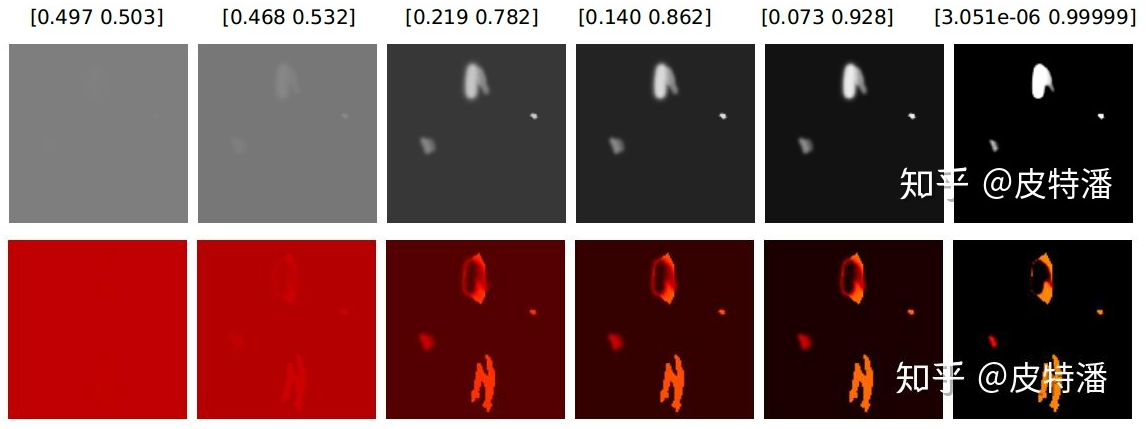• 上图是模型输出概率分布及 ce loss 的梯度，假设 $a^L$ 是模型输出，y 是 gt 值，其损失及损失对模型输出的梯度为

\begin{aligned}L &=-(y\log a^L+(1-y)\log(1-a^L)) \\ \frac{\partial J}{\partial z^L}&=\frac{\partial J}{\partial a^L}\partial a^L\\ &=(-\frac{y}{a^2}+\frac{1-y}{1-a^2}a^L(1-a^L)\\ &=-y(1-a^2)+(1-y)a^L\\ &=a^L-y\end{aligned}

• 背景有梯度：由图可知，ce loss 下背景位置也有梯度累积，尤其是在训练前期，背景梯度占据主导地位

• 正负样本平等：同样的预测概率，ce loss 正负样本贡献的梯度一样

# 交叉熵损失 (CELoss) 、二值化交叉熵损失 (BCELoss) 对权重的梯度分析？

• 输入 (x)+权重 (w)=输出（z），以上已经分析得出两个损失函数对模型输出的梯度为

$\begin{array}{rcl}\frac{\partial J}{\partial z}= &=&a-y\end{array}$

• 后续针对输出+权重的计算方式求得损失对权重的梯度，已知 z=wx+b，那么

\begin{aligned}\frac{\partial C}{\partial w_j}=\frac{1}{N}\sum_{i=1}^N(\sigma(z)-y)x_j=\frac{1}{N}\sum_{i=1}^N(a-y)x_j\\ \\ \frac{\partial C}{\partial b}=\frac{1}{N}\sum_{i=1}^N(\sigma(z)-y)=\frac{1}{N}\sum_{i=1}^N(a-y)\end{aligned}

• 注意：虽然交叉熵不计算背景点位的损失，但是求损失对权重梯度时，背景部分有共享梯度，这是因为根据链式求导 损失->激活->输出 (权重计算得到)时，激活函数在背景上有梯度回传

# 交叉熵 (CE) 与均方误差（MSE）的区别？

• 均方误差（MSE）的梯度：已知 MSE 的计算方式是 $C=(y-a)^2/2$，其中 y 是期望输出，a 是实际输出，z=wx+b，a=sigmoid (z)，则梯度为

\begin{aligned}\frac{\partial C}{\partial w}=(a-y)\sigma'(z)x=(a-y)a(1-a)x\approx a\sigma'(z)\\ \frac{\partial C}{\partial b}=(a-y)\sigma'(z)=(a-y)a(1-a)\approx a\sigma'(z)\end{aligned}

• 交叉熵 (CE)的梯度：还是使用 sigmoid 输出，然后使用 BCE 计算损失，其梯度如下

\begin{aligned}\frac{\partial C}{\partial w_j}=\frac{1}{N}\sum_{i=1}^N(\sigma(z)-y)x_j=\frac{1}{N}\sum_{i=1}^N(a-y)x_j\\ \\ \frac{\partial C}{\partial b}=\frac{1}{N}\sum_{i=1}^N(\sigma(z)-y)=\frac{1}{N}\sum_{i=1}^N(a-y)\end{aligned}

• 可知，对于 MSE，由于 $\sigma'(z)$ 在大部分区域内取趋向 0 的值，导致梯度较小，网络更新慢；CE 的w 的梯度公式中原来的 $\sigma'(z)$ 被消掉了，权重的更新是受（a-y）这一项影响（表示真实值和输出值之间的误差），即受误差的影响，所以当误差大的时候，权重更新就快，当误差小的时候，权重的更新就慢

# 神经网络输出激活函数与损失的搭配使用？

•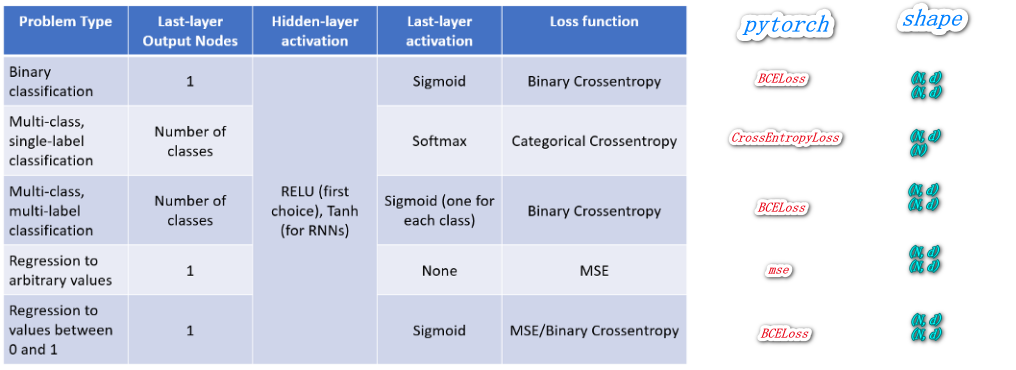• 交叉熵损失(CrossEntropyLoss)：内部将input做了softmax后再与label进行交叉熵，网络输出搭配torch.nn.Linear(previous_layer_output_num, num_classes)
• 二值交叉熵损失BCELoss：内部啥也没干，直接将input与label做了交叉熵，网络输出搭配torch.sigmoid
• BCEWithLogitsLoss：内部将input做了sigmoid后再与label进行交叉熵，网络输出torch.nn.Linear(previous_layer_output_num, num_classes)

# 当我们有 C 个目标需要分割时，模型应该如何输出 C 个类别预测还是 C+1 个？

• 交差熵指出当模型输出是 C 个类别时，目标 (gt)上的值必须是[0, C)之间的，但是由于要考虑背景输出，所以目标 (gt)一定有 C+1 个类别，所以模型输出必须是 C+1

# 什么是Dice loss?

•• dice loss 来自 dice-coefficient，dice-coefficient 是一种评估两个样本相似性的度量函数，取值范围在0到1之间，取值越大表示越相似。dice loss 是一种区域相关的 loss。意味着某像素点的 loss 以及梯度值不仅和该点的 label 以及预测值相关，和其他点的 label 以及预测值也相关 (看分母)，这点和 ce loss (交叉熵)不同，定义如下： $$DiceLoss=1-dice=1- \frac{2|X \cap Y|}{|X|+|Y|}=1-\frac{2 TP}{2 TP+FP+FN}=1-F1score$$ |X|和|Y|分别表示 X 和 Y 的元素个数. 其中分子中的系数2，是因为分母存在重复计算 X 和 Y 之间的共同元素的原因。并且 dice coefficient 是等同 F1 score，优化 dice 等同于优化 F1 score

$|\mathrm{X} \cap \mathrm{Y}|=\left[\begin{array}{llll}0.01 & 0.03 & 0.01 & 0.03 \\0.02 & 0.02 & 0.02 & 0.02 \\0.85 & 0.85 & 0.86 & 0.86 \\0.95 & 0.95 & 0.96 & 0.96\end{array}\right] *\left[\begin{array}{cccc}0 & 0 & 0 & 0 \\0 & 0 & 0 & 0 \\1 & 1 & 1 & 1 \\1 & 1 & 1 & 1\end{array}\right] \Rightarrow\left[\begin{array}{cccc}0 & 0 & 0 & 0 \\0 & 0 & 0 & 0 \\0.85 & 0.85 & 0.86 & 0.86 \\0.95 & 0.95 & 0.96 & 0.96\end{array}\right] =7.24$

• Dice loss 对正负样本严重不平衡的场景有着不错的性能，训练过程中更侧重对前景区域的挖掘。但训练 loss 容易不稳定，尤其是小目标的情况下。另外极端情况会导致梯度饱和现象。因此有一些改进操作，主要是结合 ce loss 等改进，比如: dice+ce loss，dice + focal loss 等

# dice loss 的梯度分析？

•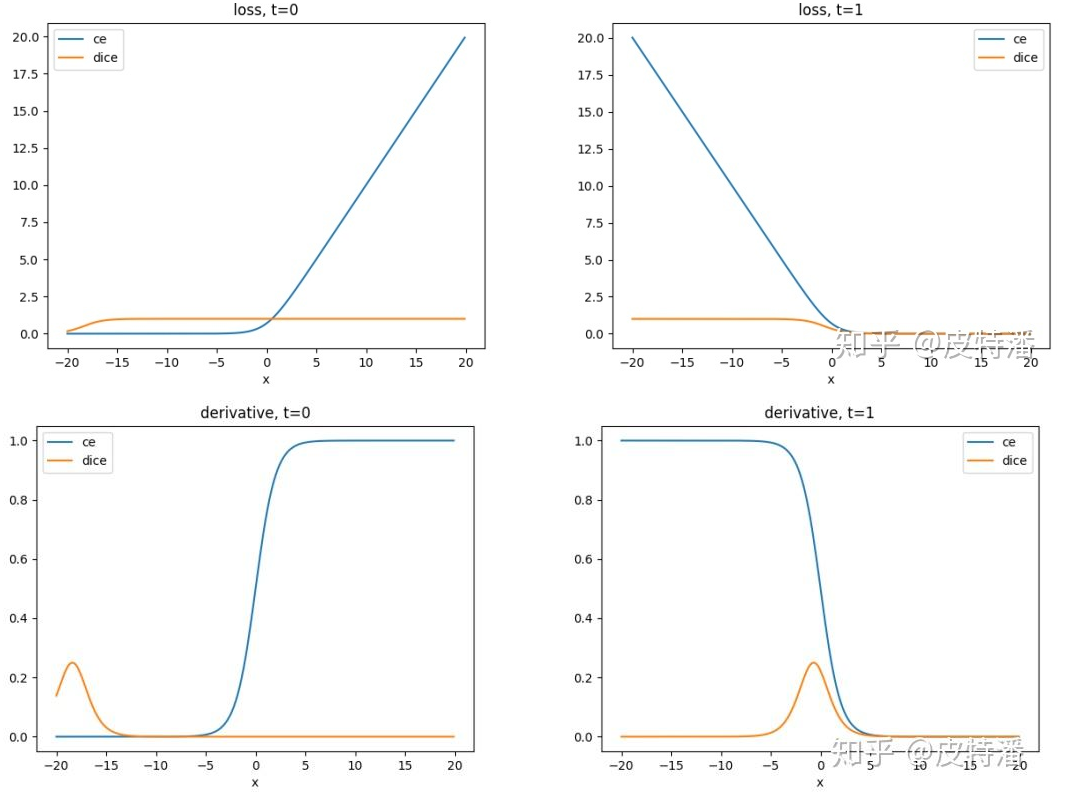• 计算输出：假设只考虑 sigmoid 输出的二分类问题，求某一点的损失函公式如下，其中 t 是 gt，y=sigmoid (x) ，

$L_{dice}=1-\frac{2ty+\varepsilon}{t+y+\varepsilon}=\begin{cases}\frac{y}{y+\varepsilon}&\text{t=0}\\ \frac{1-y}{1+y+\varepsilon}&\text{t=1}\end{cases}$

• 计算梯度

\begin{aligned}{l}\frac{dL_{dice}}{dy}&=-\frac{2t\left(t+y+\varepsilon\right)-2ty-\varepsilon}{\left(t+y+\varepsilon\right)^2}=\begin{cases}\frac{\varepsilon}{\left(y+\varepsilon\right)^2}&\text{t=0}\\ -\frac{2+\varepsilon}{\left(1+y+\varepsilon\right)^2}&\text{t=1} \end{cases} \\ \frac{dL_{dice}}{dx}&=\frac{dL_{dice}}{dy}\frac{dy}{dx}\end{aligned}

• 分析：1）当 t=0 时，同样在 x 的正常范围内， x 的梯度值接近 0；2）当 t=1 时， t 在0点附近存在一个峰值，此时 t 接近0.5。随着预测值 t 越接近1或0，梯度越小，出现梯度饱和的现象。下图上下行是不同的输出概率 heatmap 和梯度，可以看出，梯度一开始就集中在前景上，对背景有忽视作用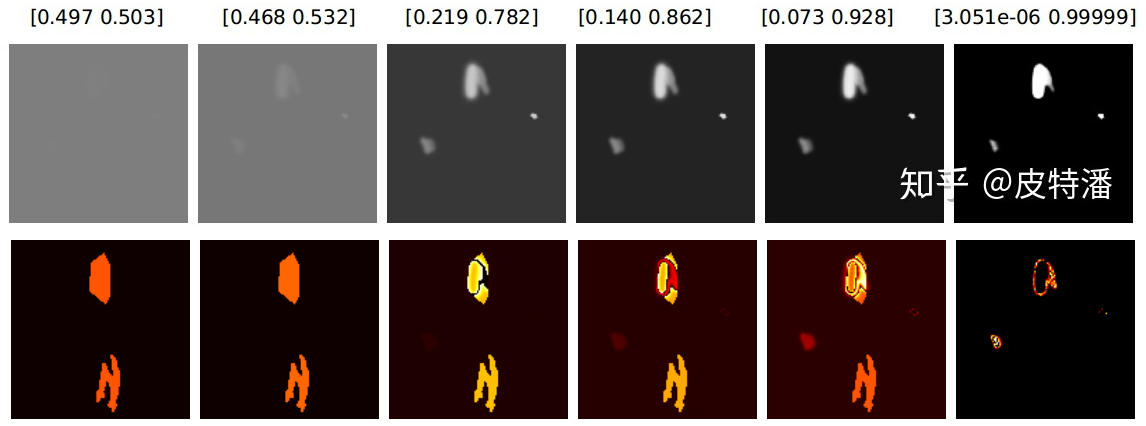# dice loss 和交叉熵的区别？

•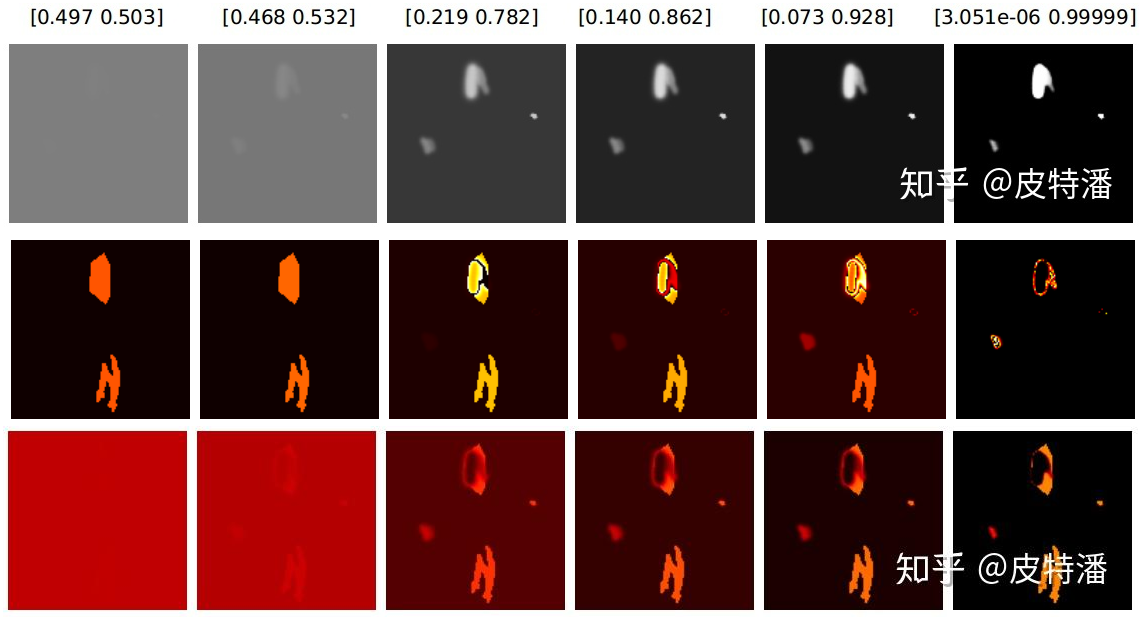• 上图从上到下，分别是模型输出概率、dice loss 、ce loss 损失的梯度
• 正负样本处理：dice loss 、ce loss 均有背景梯度，但是，dice loss 正样本的梯度值相对于 ce loss，颜色更亮，值更大，尤其是刚开始网络预测接近0.5的时候，说明 dice loss 对挖掘正样本更加有优势。ce loss 会公平处理正负样本，当出现正样本占比较小时，就会被更多的负样本淹没
• 结合使用：极端情况下，网络预测接近0或1时，对应点梯度值极小，dice loss 存在梯度饱和现象。此时预测失败 (FN，FP)的情况很难扭转回来（因为错误的点不贡献梯度，所以网络很难扭转回来）。因此结合 ce loss 使用，比如: dice+ce loss，dice + focal loss 等

# 什么是负对数似然损失(NLLLoss)？

• 1）极大似然估计(MLE) 通过寻找一组参数，最大化观测值的概率，这是已知数据出现概率的连乘，然后求偏导得到的
• 2）为了把积变和，对连乘取对数
• 3）然后在前面加负号，使得最大似然值变为最小对数似然
• 对参数的不同假设，可以得到不同数据出现的概率，也出现不同的负对数似然函数，如：高斯负对数似然损失、Poisson 负对数似然损失

# 什么是泊松负对数似然损失(PoissonNLLLoss)？

• 真实标签服从泊松分布的负对数似然损失，神经网络的输出作为泊松分布的参数λ

• 对于包含N个样本的batch数据 D( x , y )，x是预测标签，y是真实类别标签，服从泊松分布。x与y的维度相同

$\mathrm{P}(\mathrm{Y}=\mathrm{k})=\frac{\lambda^{\mathrm{k}}}{\mathrm{k} !} \mathrm{e}^{-\lambda}$

• 若x是神经网络的输出，且未进行归一化和对数化处理。第n个样本对应的损失ln为：

\begin{aligned} \mathrm{P}\left(\mathrm{Y}=\mathrm{y}_{\mathrm{n}}\right)=\frac{x_{\mathrm{n}}^{\mathrm{y}_{\mathrm{n}}}}{\mathrm{y}_{\mathrm{n}} !} \mathrm{e}^{-\mathrm{x}_{\mathrm{n}}} \\ 1_{\mathrm{n}}=-\log \mathrm{P}\left(\mathrm{Y}=\mathrm{y}_{\mathrm{n}}\right)=\mathrm{x}_{\mathrm{n}}-\mathrm{y}_{\mathrm{n}} \log \mathrm{x}_{\mathrm{n}}+\log \left(\mathrm{y}_{\mathrm{n}} !\right) \end{aligned}

# 什么是高斯数似然损失(GaussianNLLLoss)？

• 真实标签服从高斯分布的负对数似然损失，神经网络的输出作为高斯分布的均值和方差
• 对于包含N个样本的batch数据D(x,var,y)，x神经网络的输出，作为高斯分布的均值，var神经网络的输出，作为高斯分布的方差，y是样本对应的标签，服从高斯分布。x与y的维度相同，var和x的维度相同
• 第n个样本对应的损失ln为

$l_{n}=0.5 *\left(\log \left(\max \left(\operatorname{var}_{\mathrm{n}}, \mathrm{eps}\right)\right)+\frac{\left(\mathrm{x}_{\mathrm{n}}-\mathrm{y}_{\mathrm{n}}\right)}{\max \left(\operatorname{var}_{\mathrm{n}}, \mathrm{eps}\right)}\right)$

# 什么是MarginRankingLoss ？

• 计算输入input，other 和 标签label间的 margin rank loss 损失

$margin\_rank\_loss = max(0, -label * (input - other) + margin)$

# 什么是MultiLabelMarginLoss？

• 对于mini-batch(小批量) 中的每个样本

# 什么是SoftMarginLoss？

• $\mathrm{loss}(x,y) = \sum_i \dfrac{\log(1 + \exp (-y[i] \cdot x[i]))}{x.\mathrm{nelement()}}$

• $x.\mathrm{nelement()}$为输入x中的样本个数。注意这里y也有1和-1两种模式

# 什么是MultiLabelSoftMarginLoss？

• 多标签 one-versus-all 损失

# 什么是HingeEmbeddingLoss？

• 测量输入张量的损耗x 和一个标签张量y （包含1或-1）。通常用于测量两个输入是否相似或不相似，例如使用L1成对距离作为x ，通常用于学习非线性嵌入或半监督学习

$l_{n}= \begin{cases}x_{n}, & \text { if } y_{n}=1 \\ \max \left\{0, \Delta-x_{n}\right\}, & \text { if } y_{n}=-1\end{cases}$

# 什么是CosineEmbeddingLoss？

• $\operatorname{loss}(x, y)=\left\{\begin{array}{ll} 1-\cos \left(x_{1}, x_{2}\right), & \text { if } y==1 \\ \max \left(0, \cos \left(x_{1}, x_{2}\right)-\operatorname{margin}\right), & \text { if } y==-1 \end{array}\right.$

# 什么是三元损失TripletMarginLoss？

• 就构成了一个三元组，学习目标是让Positive和Anchor之间的距离 D ( a , p )尽可能的小，Negative和Anchor之间的距离 D ( a , n )尽可能的大

$L=\sum_{i}^{N}\left[\left\|f\left(x_{i}^{a}\right)-f\left(x_{i}^{p}\right)\right\|_{2}^{2}-\left\|f\left(x_{i}^{a}\right)-f\left(x_{i}^{n}\right)\right\|_{2}^{2}+\alpha\right]_{+}$

# 什么是CTCLoss？

• 连接时序分类损失，可以对没有对齐的数据进行自动对齐，主要用在没有事先对齐的序列化数据训练上。比如语音识别、ocr识别等等

# 什么是0-1损失？

• 计算 0-1 分类损失的总和或平均值

$L(Y, f(x)) = \begin{cases} 1,& Y\ne f(x)\\ 0,& Y = f(x) \end{cases}$

• 当且仅当预测为真的时候取值为1，否则取值为0。该损失函数能够直观的刻画分类的错误率，但是由于其非凸、非光滑的特点，使得算法很难直接对该函数进行优化

# 逻辑斯谛损失 (Logistic Loss)？

• 又称对数损失

$L(\hat y,y)=-(ylog(\hat y)+(1-y)log(1-\hat y))$

• Logistic损失函数也是0-1损失函数的凸上界，且该函数处处光滑，因此可以使用梯度下降法进行优化。但是，该函数对所有样本点都做惩罚，因此对异常点更为敏感

# 什么是指数损失 (Exponential Loss)？

$L(Y, f(x)) = \exp(-Yf(x))$

# 什么是对数损失(Logarithmic Loss)?

• 又称二元交叉熵损失，主要用于逻辑回归算法（Logistric Regression）

$loss=-\frac {1} {N} \sum_{i=1}^My_{ij}log(p_{ij})$

• 这里N是样本的数量，M是类别数量，yij和pij都是二值型标志位，表示第i个样本是否属于第j类，y表示真实值，p表示预测值。logloss越小说明算法越好。在实际编程应用中注意添加一个冗余项(1e−15)之类的，避免出现log0这样的情况

# 对数损失函数和极大似然估计有什么关系？

• 对数损失函数等价于极大似然估计(MLE)
• 似然估计：一组参数在一堆数据下的似然值，等于每一条数据在这组参数下的条件概率之积
• 对数损失：而损失函数一般是每条数据的损失之和，为了把积变为和，就取了对数
• 对数损失加负号：让最大似然值和最小损失对应起来

# 什么是合页损失(铰链损失) (Hinge Loss)?

• 也称为铰链损失，用于分类的损失函数，旨在找到距离每个训练样本都尽可能远的决策边界(decisionboundary)，从而使样本和边界之间的裕度最大化，即最大间隔算法。其中$y_i(wx_i+b)$为点$x_i$到超平面(w,b)的函数间隔

\left\{ \begin{aligned} sample_{pos}:w*x_i+b>=1 \\ sample_{neg}:w*x_i+b<=-1 \end{aligned} \right. \Rightarrow y_i(wx_i+b)>=1 \\ \underset{\min}{w,b}\sum_{i=1}^N (1-y_i(wx_i+b))+\lambda\Vert w\Vert ^2

• 由于Hinge损失在 $y_i(w*x_i+b)$处不可导，因此不能使用梯度下降算法优化，而是使用次梯度下降法

# 什么是修正huber损失?

• 如果$y_i f(x_i) > 1$，则$L(y_i, f(x_i)) = \max(0, 1 - y_i f(x_i))^2$ ,否则，$L(y_i, f(x_i)) = -4 y_i f(x_i)$

# 什么是Brier损失(Brier score loss)?

• 计算二分类的Brier分数

$BS = \frac{1}{n_{\text{samples}}} \sum_{i=0}^{n_{\text{samples}} - 1}(y_i - p_i)^2$

• Brier score loss也在0到1之间，值越低（均方差越小），预测越准确

# 什么是标签排名损失(label rank loss)?

• 该损失对样本中错误排序的标签对的数量进行平均，即真实标签的分数低于虚假标签，加权为虚假标签和真实标签的有序对数量的倒数

# 什么是内聚外斥损失(Center Loss) ？

•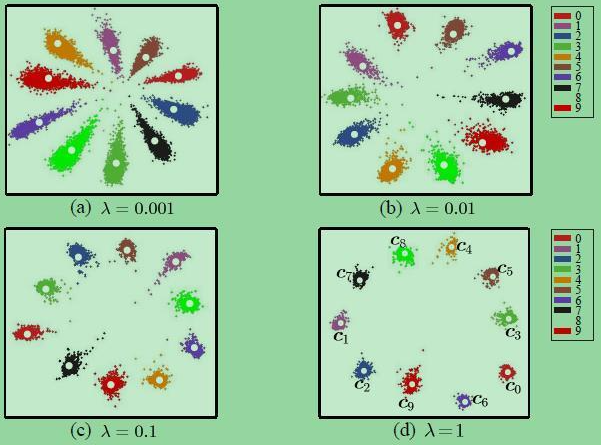• 在分类任务中， Cross Entropy Loss (交叉熵代价) + Softmax 组合被广泛应用，可以理解为：更相似(同类/同物体)的图像在特征域中拥有“更近的距离”, 相反则”距离更远“，Softmax损失能够要求特征具有类间的可分离性，但不能约束特征的类内紧凑性，因此Center Loss被提出解决这个问题

• 计算公式

$L = L_{S} + \lambda L_{C} \\ = -\sum^{m}_{i=1}log\frac{e^{W_{y}^{T}x_{i}+b_{y_{i}}}}{\sum^{m}_{i=1}e^{W^{T}_{j}x_{i}+b_{j}}} + \frac{\lambda}{2}\sum^{m}_{i=1}||x_{i}-c_{y_{i}}||^{2}_{2}$

• 如图是softmax加center Loss前后的效果，可以看出，类间距离变大了，类内距离减少了

# 什么是IOU loss?

•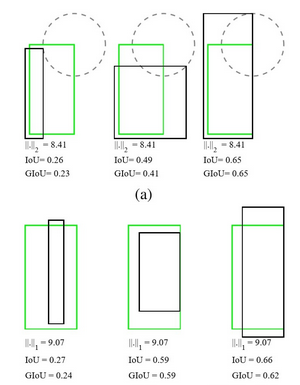• 目标检测算法检测评价使用 IoU，但实际回归的是坐标框4个坐标点，两者是不等价的，甚至绝对值损失 (L1Loss)或者平方损失 (MSELoss/L2Loss)相等时，其 IoU 不是唯一的。旷视2016年提出 IoU Loss，其将4个点构成的 box 看成一个整体进行回归 (以下2种定义均可) $$\begin{array}{ll}Loss_{IOU}=-\ln (IOU) \ Loss_{IOU}=1-IOU\end{array}$$
• 缺点： 当预测框和真实框的 IOU>0时，其 loss 可以被算出来，但是对于哪些 IOU=0的框，无法算出 loss。导致无法区分哪些预测更加靠近真实框的结果

# 什么是GIOU loss?

•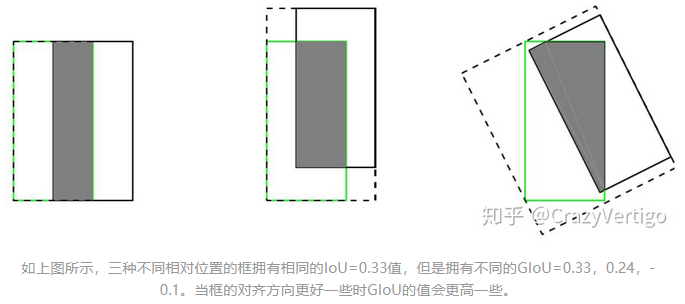• 考虑到IOU loss存在个问题：（1）无法对两个不重叠的框进行优化；（2） 无法反映出两个框到底距离有多远。IoU Loss引入了C检测框（包含真实框和检测框的最小矩形），当检测框与真实框有重叠时，GIoU等效于IOU

$Loss_{GIOU}=1-(IOU-\frac{C-(A \bigcup B)}{C})$

• 缺点： 当目标框完全包裹预测框的时候，IoU 和 GIoU 的值都一样，此时 GIoU 退化为 IoU, 无法区分其相对位置关系

# 什么是 DIOU Loss?

•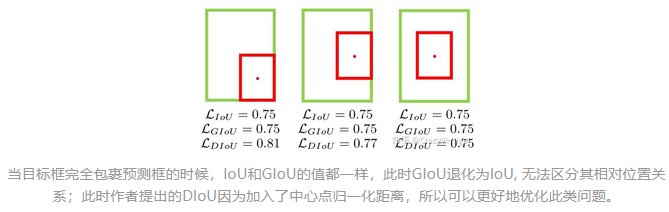• 当目标框完全包裹预测框的时候，IOU loss和GIoU loss的值都一样，此时GIoU退化为IoU, 无法区分其相对位置关系，此时作者提出的DIoU因为加入了中心点归一化距离

$L_{DIoU}=1-IoU+\frac{\rho^{2}\left(b, b^{g t}\right)}{c^{2}}$

• $b, b^{gt}$ 表示预测框和真实框中心点，$\rho$ 表示欧式距离，$c$ 表示预测框与真实框的最小外接矩形的对角线距离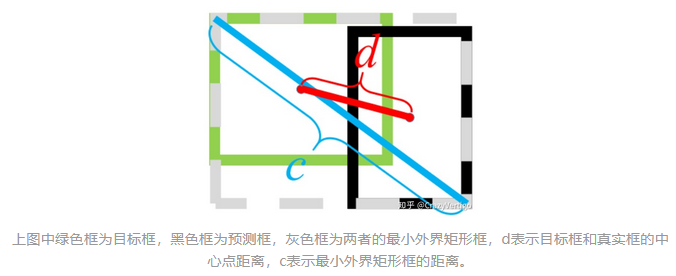# 什么是 CIOU loss？

• 在 DIou Loss 的惩罚项基础上加了一个影响因子 $\alpha v$ ，这个因子把预测框长宽比拟合目标框的长宽比考虑进去

$L_{CIoU}=1-IoU+\frac{\rho^{2}\left(b, b^{g t}\right)}{c^{2}}+\alpha v\\$

• $b,b^{gt}$ 表示预测框和真实框中心点，$\rho$ 表示欧式距离，$c$ 表示预测框与真实框的最小外接矩形的对角线距离• $\alpha$是用于做权重参数，$v$是用来衡量长宽比一致性的参数

$\alpha=\frac{v}{(1-I o U)+v}\\ v=\frac{4}{\pi^{2}}\left(\arctan \frac{w^{g t}}{h^{g t}}-\arctan \frac{w}{h}\right)^{2}$

# 什么是 EIOU loss?

•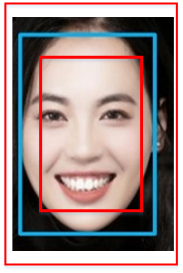• CIoU loss同时考虑到回归框宽高比例以及真实框与预测框中心距离，但是存在一个问题，他仅仅将宽高比例作为影响因子，如果说有两个框中心点与原始图保持一致，宽高比例相同但是宽高的值不同，按照CIOUloss损失可能一致与回归目标不相符

• EIOU loss将原始的宽高比例，改为宽高值回归，将红色框的宽度与真实（蓝色）宽度进行损失，红色长度与真实长度进行回归

$=1-I O U+\frac{\rho^{2}\left(\mathbf{b}, \mathbf{b}^{\mathbf{g t}}\right)}{c^{2}}+\frac{\rho^{2}\left(w, w^{g t}\right)}{C_{w}^{2}}+\frac{\rho^{2}\left(h, h^{g t}\right)}{C_{h}^{2}}$

• $b,b^{gt}$ 表示预测框和真实框中心点，$\rho$ 表示欧式距离，$c$ 表示预测框与真实框的最小外接矩形的对角线距离• $w,h$ 为预测框的宽高， $w^{gt},h^{gt}$ 为真实框的宽高， $C_w,C_h$ 为外接框的宽高

• 考虑在一张图像中回归误差小的高质量锚框的数量远少于误差大的低质量样本，质量较差的样本会产生过大的梯度影响训练过程，使用IOU值对EIOU loss进行加权，得到Focal EIOU loss

$L_{\text {Focal-EIOU }}=I O U^{\gamma} L_{E I O U}$

# 什么是 SIOU loss?

•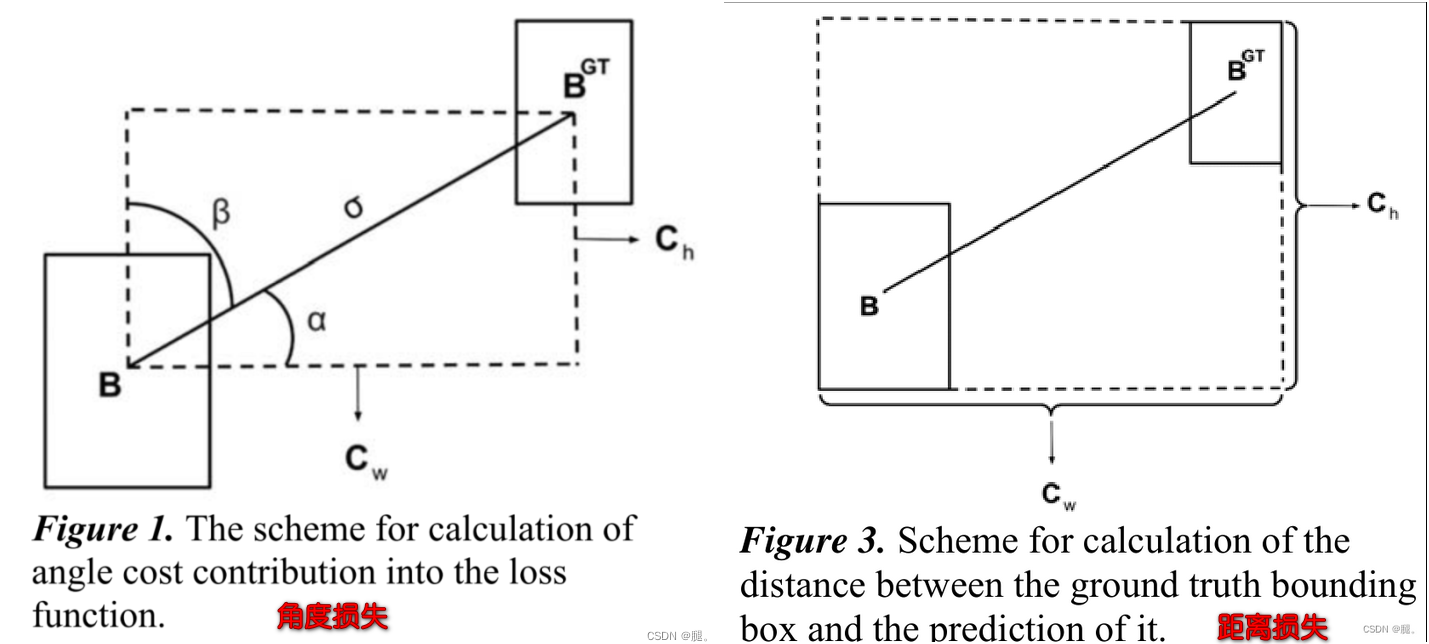• 将预测框和真实框的角度考虑进来，加快收敛，下图B是目标框，B_GT是回归框，当B到B_GT的夹角小于alpha时，向最小alpha收敛，反之向beta收敛

• SIoU =Distance cost（angle + distance）+Shape cost+IOU loss

• 角度损失 (angle loss):

$\begin{array}{c} \Lambda=1-2 * \sin ^{2}\left (\arcsin (x)-\frac{\pi}{4}\right) \\ \\ x=\frac{c_{h}}{\sigma}=\sin (\alpha) \\ \sigma=\sqrt{\left (b_{c_{x}}^{g t}-b_{c_{x}}\right)^{2}+\left (b_{c_{y}}^{g t}-b_{c_{y}}\right)^{2}} \\ c_{h}=\max \left (b_{c_{y}}^{g t}, b_{c_{y}}\right)-\min \left (b_{c_{y}}^{g t} b_{c_{y}}\right) \end{array}$

• 距离损失(Distance loss)：将角度信息考虑进行距离损失，当角度为0时，r=2，退化为距离损失

$\Delta=\sum_{t=x, y}\left(1-e^{-\gamma \rho_{t}}\right) \\ \\ \rho_{x}=\left(\frac{b_{c_{x}}^{g t}-b_{c_{x}}}{c_{w}}\right)^{2}, \rho_{y}=\left(\frac{b_{c_{y}}^{g t}-b_{c_{y}}}{c_{h}}\right)^{2}, \gamma=2-\Lambda$

• 形状损失(Shape loss)：θ为控制参数，如果θ值设置为1，将立刻优化形状，从而影响形状的自由运动

$\Omega=\sum_{t=w, h}\left(1-e^{-\omega_{t}}\right)^{\theta} \\ \\ \omega_{w}=\frac{\left|w-w^{g t}\right|}{\max \left(w, w^{gt}\right)}, \omega_{h}=\frac{\left|h-h^{g t}\right|}{\max \left(h, h^{g t}\right)}$

• IOU损失(iou loss)$1-IOU$

• 总损失(SIOU loss)： $L_{b o x}=1-IoU+\frac{\Delta+\Omega}{2}$

# 什么是标签平滑(Class label smoothing)?

•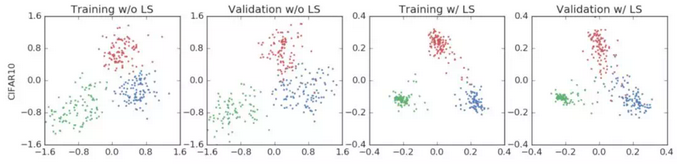• 后面两列展示，标签平滑为最终的激活产生了更紧密的聚类和更大的类别间的分离
• 对于分类问题，特别是多分类问题，常常把向量转换成独热编码（one-hot encoding），对于损失函数，我们需要用预测概率去拟合真实概率，由此带来2个问题：（1）无法保证模型的泛化能力，容易造成过拟合；（2） 全概率和0概率鼓励所属类别和其他类别之间的差距尽可能加大，而由梯度有界可知
• 对预测有信心可能表明模型是在记忆数据，而不是在学习， 平滑就是一定程度缩 label 中 min 和 max 的差距，适当让 label 两端的极值往中间靠，可以增加泛化性能

# 标签平滑(Class label smoothing) 的原理？

• 标签平滑(Class label smoothing) 目标是重构one-hot变量，平滑公式如下，其中为平滑后的标签向量，为平滑因子，为人为引入的固定分布

$y^{\prime}=(1-\epsilon) * y+\epsilon * u$

• 假设样本的 one-hot 化后的标签为[ 0 , 0 , 0 , 1 , 0 ]，仍假设我们已经得到了该样本进行 softmax 的概率矩阵 $p=\left[p_{1}, p_{2}, p_{3}, p_{4}, p_{5}\right]=[0.1,0.1,0.1,0.36,0.34]$ ，如果平滑因子

$\begin{array}{l} y_{1}=(1-\epsilon) *[0,0,0,1,0]=[0,0,0,0.9,0] \\ y_{2}=\epsilon *[1,1,1,1,1]=[0.1,0.1,0.1,0.1,0.1] \\ y=y_{1}+y_{2}=[0.1,0.1,0.1,1.0,0.1] \end{array}$

• 不使用标签平滑及使用标签平滑的损失如下计算交叉熵损失(CrossEntropyLoss) ，经过平滑后的损失变大，因为考虑了0位置的损失

$\operatorname{loss}=-y * \log p=-[0,0,0,1,0] * \log ([0.1,0.1,0.1,0.36,0.34])=1.47 \\ \operatorname{loss_{smooth}}=-y * \log p=-[0.1,0.1,0.1,1.0,0.1] * \log ([0.1,0.1,0.1,0.36,0.34])=2.63$

]]>
<p>本文讲解神经网络使用的损失，用于衡量模型输出与标签值的差异，尤其需要把握的是不同模型输出使用不同的激活函数，然后使用不同损失函数去计算</p>

# 什么是数据增强？

• 指在计算机视觉领域中对图像进行数据增强，从而弥补训练图像数据集的不足，达到对训练数据扩充的目的
• 数据增强可分为同类数据增强（如：翻转、旋转、缩放、移位、模糊等）和混类数据增强（如 mixup）两种方式

# 什么是同类、混类数据增强？

• 同类数据增强：对单张图片内的增广操作，包括翻转、旋转、缩放、移位、模糊等，torchvision 的 transforms 实现了大部分的同类数据增强
• 混类数据增强：对多幅图像进行融合，生成一幅图像

# 什么是在线、离线数据增强？

• 在线数据增强：边训练边进行数据增强，好处是生成的样本几乎是无限的，坏处是增加训练时间
• 离线数据增强：先进行数据增强再进行训练，好处是可以控制数据增强的方向，坏处是增加额外存储

# 什么是Random Erasing数据增强？

•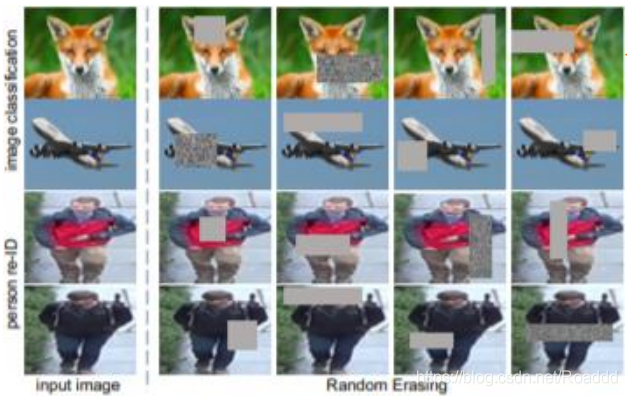• 在现实场景中，遮挡问题一直都是一个难以处理和解决的问题。为了更 好的实现对遮挡数据的模拟，利用 Random Erasing 的方式，将原数据集中一部分保持原样， 另外一部分随机擦除一个矩形区域
• 设置五个超参数，分别表示原数据集中实施 Random Erasing 的概率 P，擦除面积比 率的下界 sl 和上界 sh，擦除矩形长宽比的下界 r1 和上界 r2
• 首先通过均匀分布得到一个概率 p1，如果满足条件，实施擦除
• 擦除的过程如下：通过均匀分布取样得到擦除矩形面积，以及长宽值。选择一个满 足所有矩形部分都在图像内的左上角坐标，将这个矩形区域都设置为统一和图像其 他区域无关的纯色值

# 什么是HideAndSeek数据增强？

•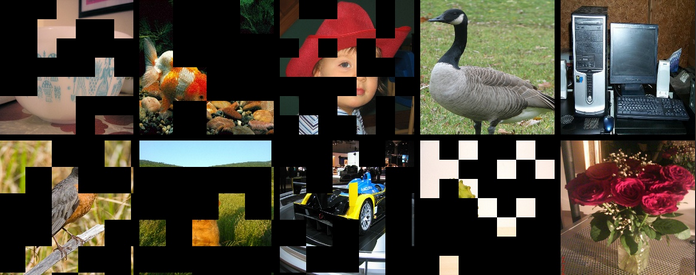• 将图像分为若干块区域(patch)，对于每块区域，都以一定的概率生成掩码，不同区域的掩码含义如图

•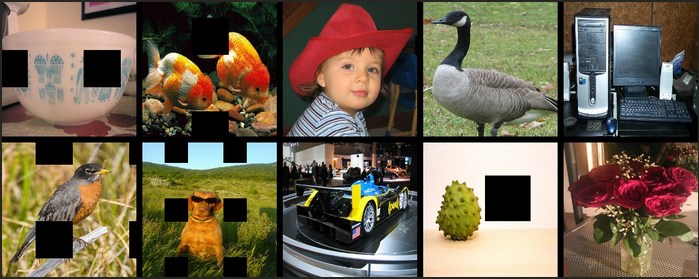• 过度删除或过度保留成为需要解决的核心问题。过度删除区域可能造成目标主体大部分甚至全部被删除，或者导致上下文信息的丢失，导致增广后的数据成为噪声数据；过度保留，对目标主体及上下文基本产生不了什么影响，失去增广的意义
• 通过生成一个与原图分辨率相同的掩码，并将掩码进行随机翻转，与原图相乘，从而得到增广后的图像，通过超参数控制生成的掩码网格的大小， 避免过度删除或过度保留问题

# 什么是Cutout数据增强？

•• Cutout的出发点和 Random Erasing一样，也是模拟遮挡，目的是提高泛化能 力，实际上比 Random Erasing 简单，随机选择一个固定大小的正方形区域，然后采用全 0 填充
• Cutout 区域的大小比形状重要，所以 Cutout 只要是正方形就行。具体操作是利用固定大小的矩形 对图像进行遮挡，在矩形范围内，所有的值都被设置为 0，或者其他纯色值，而且擦除矩形 区域存在一定概率不完全在原图像中（文中设置为 50%）
• Cutout 可以理解为 Dropout 的一种扩展操作，不同的是 Dropout 是对图像经过网络后生成的特征进行遮挡，而 Cutout 是直接对输入的图像进行遮挡，相对于Dropout，Cutout 对噪声的鲁棒性更好

# 什么是Mixup数据增强？

•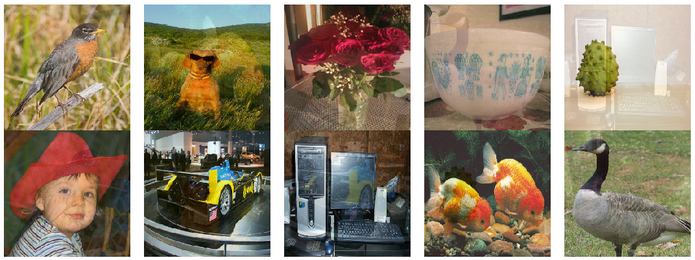• Mixup 是最先提出的图像混叠增广方案，其原理简单、方便实现，不仅在图像分类上，在目标检测上也取得了不错的效果。
• 为了便于实现，通常只对一个 batch 内的数据进行混叠，即随机选取两张样本按比例混合，分类的结果按比例分配
• 注意：（1）不同类别的图像mix效果会更好；(2) 主要用于分类

# 什么是CutMix数据增强？

•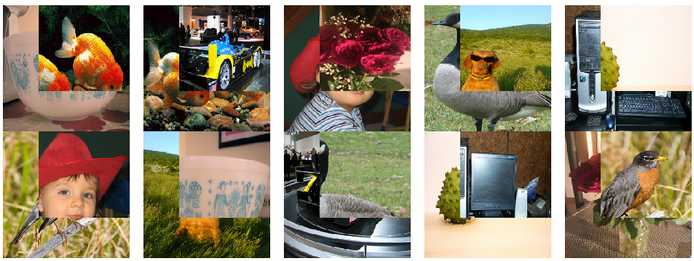• 与Mixup直接对两幅图进行相加不一样，Cutmix是从一幅图中随机裁剪出一个ROI，然后覆盖当前图像中对应的区域，分类结果按一定的比例分配
• 效果等于：Cutout + Mixup

# 什么是Mosaic数据增强？

•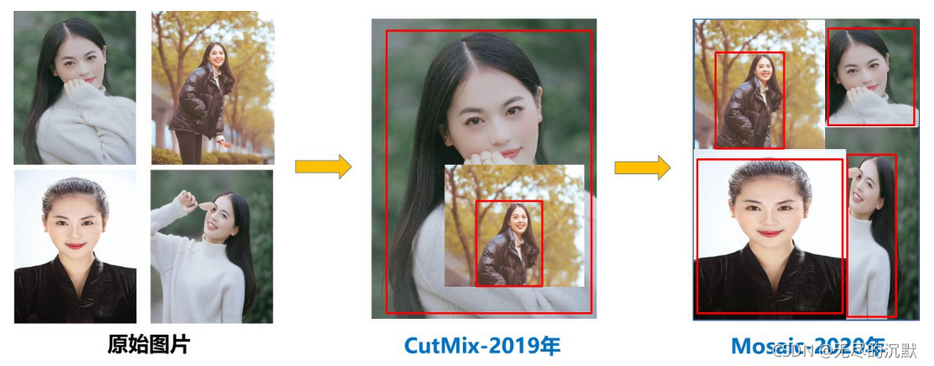• YOLOv4的Mosaic 数据增强参考了CutMix 数据增强方式，利用了四张图像，然后随机缩放、随机裁剪、随机排布的方式进行拼接
• 论文中描述其 拥有一个巨大的优点就是丰富检测物体的背景，且在BN 计算的时候一下子会计算四张图片的数据

# Mixup、Cutout、CutMix区别?

• Cutout 和 CutMax 就是填充区域像素的区别
• Mixup 和 CutMix 是混合两种样本方式上的区别：Mixup 是将两张图按比例进行插值来混合样本，CutMix 是采用 Cut 部分区域再补丁的形式去混合图像，不会有图像混合后不自然的情形

• 一种新的数据增强方法，可以一定程度上抵抗对抗攻击。其包括两个阶段，每个阶段进行一次前向传播和一次反向传播
• 前向传播： 为了最小化成本函数，神经网络改变原始图像而不是网络权重。通过这种方式，神经网络对自己执行对抗性攻击，改变原始图像以产生图像上没有所需对象的欺骗
• 反向传播： 神经网络被训练以正常方式检测此修改图像上的物体

# 什么是测试时增强(Test-Time-Augmentation，TTA)?

• 数据增强同样也可以应用于测试阶段，将原始的图像进行各种形式的变换得到多张图片，比如图像翻转，图像旋转，图像拉伸等操作，然后对这些图片分别进行推理，再对这多个结果进行综合分析以确定最终结果，这便是测试时增强
• 通过分析多项数据增强的图像，然后综合分析，有可能会平滑掉某一种变换导致的关键信息丢失现象带来的损失，从而提升预测的准确率
]]>
<p>本文介绍数据增强的基础概念，并对对复杂的混类数据增强作了原理介绍</p>

# 什么是迁移学习 (transfer learning)?

• 将知识从较简单任务的解决方案迁移到较复杂的任务，或者将知识从数据较多的任务迁移到数据较少的任务，大多数机器学习系统都只能完成一项任务
• 迁移学习的核心是：找到源领域和目标领域之间的相似性，并加以合理利用。这种相似性非常普遍。比如，不同人的身体构造是相似的；自行车和摩托车的骑行方式是相似的；国际象棋和中国象棋是相似的；羽毛球和网球的打球方式是相似的
• 迁移学习是迈向人工智能的一小步；在人工智能中，单个程序可以完成多项任务

# 为什么需要迁移学习？

• 大数据与少标注的矛盾：虽然有大量的数据，但往往都是没有标注的，无法训练机器学习模型。人工进行数据标定太耗时
• 大数据与弱计算的矛盾：普通人无法拥有庞大的数据量与计算资源。因此需要借助于模型的迁移
• 普适化模型与个性化需求的矛盾：即使是在同一个任务上，一个模型也往往难以满足每个人的个性化需求，比如特定的隐私设置。这就需要在不同人之间做模型的适配

# 什么是负迁移?

• 在源域上学习到的知识，对于目标域上的学习产生负面作用
• 可能是数据问题导致，即源域和目标域压根不相似；也可能是方法问题，虽然源域和目标域是相似的，但是，迁移学习方法不够好，没找到可迁移的成分

# 什么是基于样本迁移？

•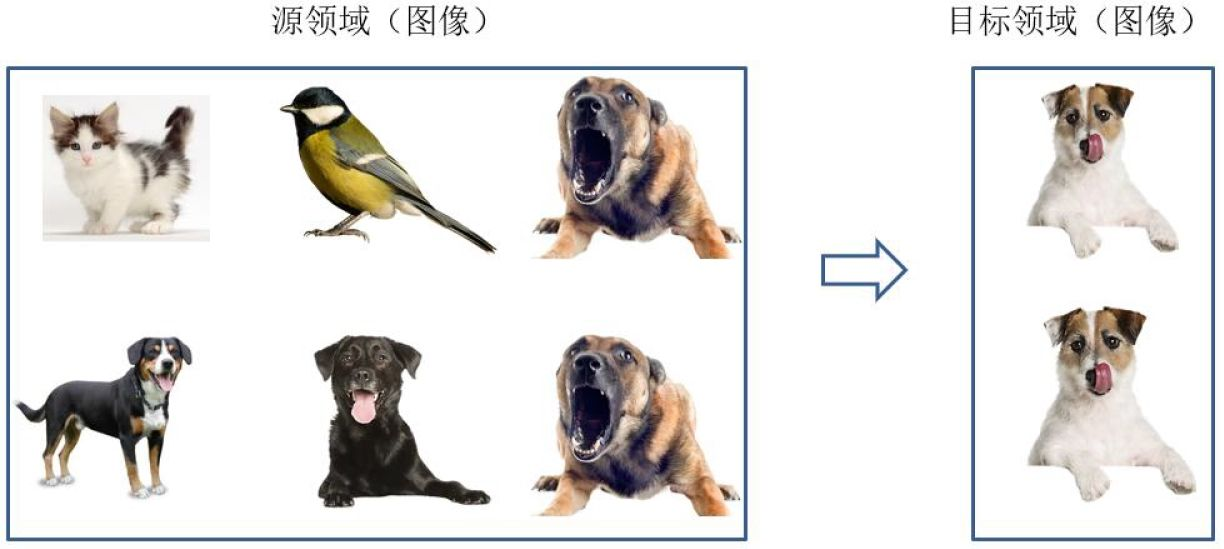• 根据一定的权重生成规则，对数据样本进行重用，来进行迁移学习
• 上图表示了基于样本迁移方法的思想源域中存在不同种类的动物，如狗、鸟、猫等，目标域只有狗这一种类别。在迁移时，为了最大限度地和目标域相似，我们可以人为地提高源域中属于狗这个类别的样本权重

# 什么是基于特征迁移？

•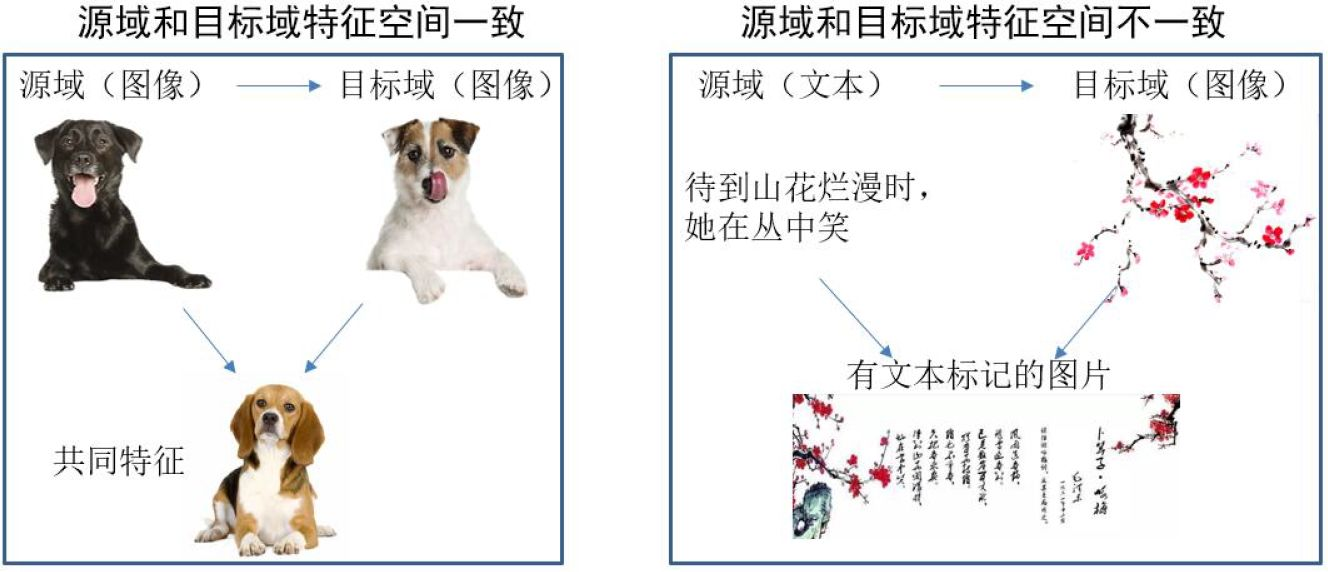• 通过特征变换的方式互相迁移,来减少源域和目标域之间的差距；或者将源域和目标域的数据特征变换到统一特征空间中,然后利用传统的机器学习方法进行分类识别。根据特征的同构和异构性,又可以分为同构和异构迁移学习

# 什么是基于模型迁移？

•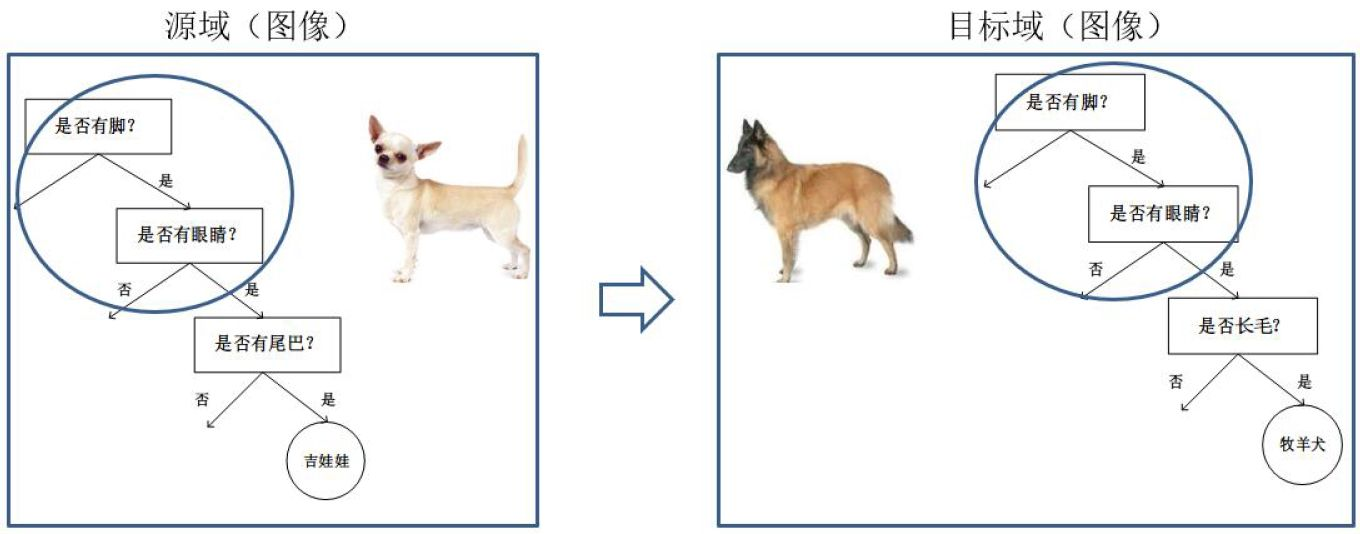• 指从源域和目标域中找到他们之间共享的参数信息,以实现迁移的方法。这种迁移方式要求的假设条件是： 源域中的数据与目标域中的数据可以共享一些模型的参数

# 什么是基于关系迁移？

•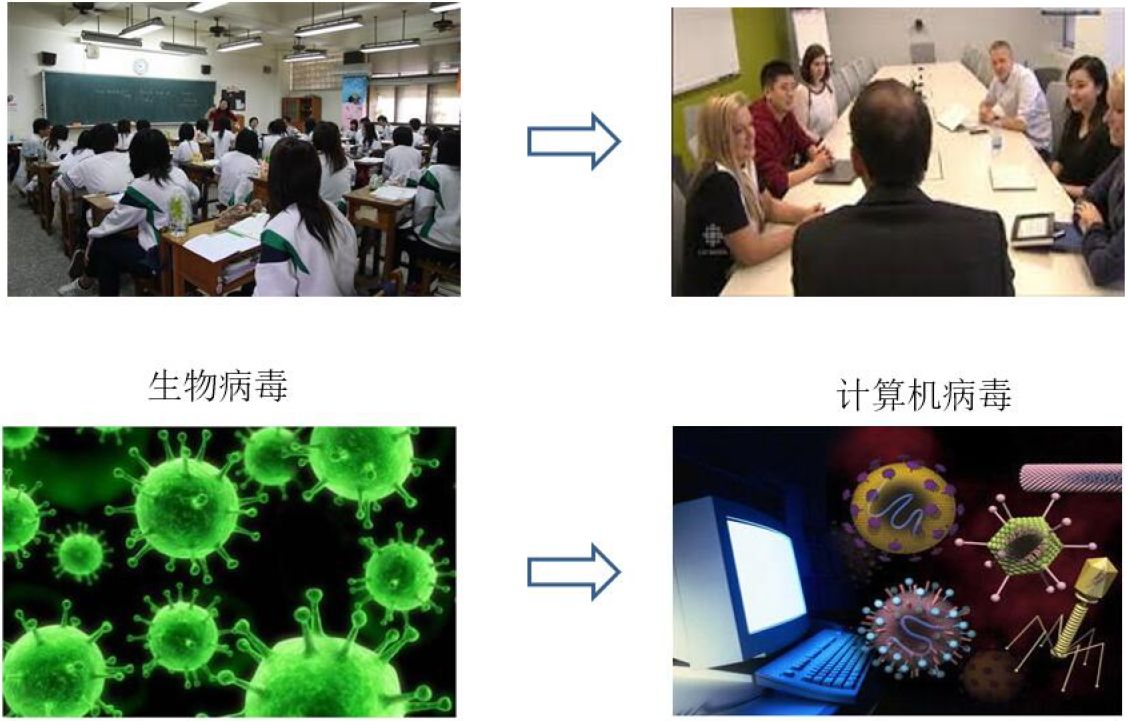• 这种方法比较关注源域和目标域的样本之间的关系

# 什么是模型微调 (fine tune)？

• 指利用别人己经训练好的网络，针对自己的任务再进行调整，是迁移学习的一种实现方式。微调和从头训练（train from scratch）的本质区别在于模型参数的初始化
• 从头训练(train from scratch)：通常指对网络各类参数进行随机初始化（当然随机初始化也存在一定技巧），随机初始化模型通常不具有任何预测能力，通常需要大量的数据或者特定域的数据进行从零开始的训练，这样需要训练到优秀的模型通常是稍困难的
• 微调初始化：网络各类参数已经在其他数据集（例如ImageNet数据集）完成较好调整的，具备了较优秀的表达能力。因此，我们只需要以较小的学习速率在自己所需的数据集领域进行学习即可得到较为优秀的模型。微调通常情况下，无须再重新设计网络结构，预训练模型提供了优秀的结构，只需稍微修改部分层即可。在小数据集上，通常微调的效果比从头训练要好很多，原因在于数据量较小的前提下，训练更多参数容易导致过度拟合

# fine-tuning 模型的三种状态?

• 状态一：只预测，不训练
• 状态二：训练，但只训练最后分类层
• 状态三：完全训练，分类层+之前卷积层都训练

# 迁移学习与传统机器学习有什么区别？

•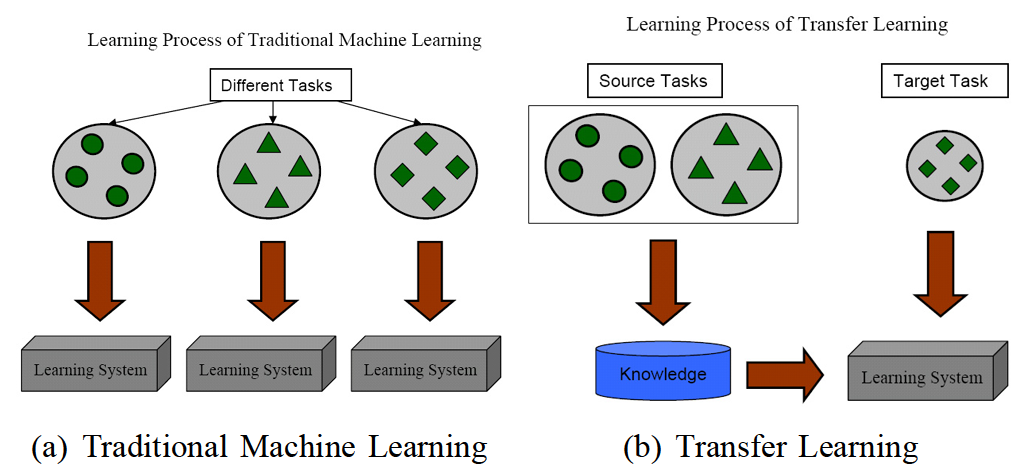• 迁移学习：(1) 训练和测试数据不需要同分布；(2) 不需要足够的数据标注；(3)可以重用之前的模型
• 传统机器学习：（1）训练和测试数据同分布；(2) 足够的数据标注；(3)每个任务分别建模
]]>
<p>本文介绍迁移学习的基本概念，迁移时需要关注从源域拿来什么“知识”，以使得目标域不是从0开始学习</p>

# 什么是batch size?

• 在神经网络的训练过程中的超参数，决定在一次训练中，要选取多少样本喂给神经网络，这个要选择的样本个数，就是batch size

# batch size大小对网络训练的影响？

• 合理增大： 加速网络训练速度，提高了内存利用率，大矩阵乘法并行计算效率提高；在一定范围内，一般来说 Batch_Size 越大，其确定的下降方向越准，震荡比较小
• 不合理增大：内存利用率提高了，但是内存容量可能撑不住了
• 不合理减小： 会导致每次计算的梯度不稳定，引起训练的震荡比较大，很难收敛
• Batch_Size 增大到某个时候，达到时间上的最优：因为随着batchsize增大，处理相同的数据量的速度越快，并且达到相同精度所需要的epoch数量越来越多

# 为什么10000样本训练1次会比100样本训练100次收敛慢呢？

• 假设样本真实的标准差为$\sigma$，则 n 个样本均值的标准差为$\frac {\sigma} {\sqrt{n}}$ ,$\sqrt{n}$表明使用更多样本来估计梯度的方法回报是低于线性的
• 10000个样本训练一次和100个样本训练一次，由于计算量是线性的，前者的计算量是后者的100倍，但均值标准差只比后者降低了10倍，那么在相同的计算量下（同样训练10000个样本），小样本的收敛速度是远快于使用整个样本集的

# 如何选择合理的batch size?

• 更大的batch size会得到更精确的梯度估计值，但其估计梯度的回报是低于线性的
• 如果训练集较小，可以直接使用梯度下降法，batch size等于样本集大小
• 在某些硬件上使用特定大小的数组时，运行时间会更少。尤其是在使用 GPU 时，通常使用2的幂数作为 batch size 可以获得更少的运行时间，通常10到100，当有足够算力时，选取32,64,128或更小一些的 batch_size。算力不够时，在效率和泛化性之间做权衡，选择更小的 batch size

# 什么是参数初始化?

• 在网络模型训练之前，对各个节点的权重和偏置进行初始化赋值的过程
• 目的： 防止模型在通过深度神经网络的正向传递过程中爆炸或消失。如果发生这种情况，损失梯度将太大或太小而无法向后流动，并且网络将需要更长的时间来收敛

# 理想的参数初始化应该满足什么条件？

•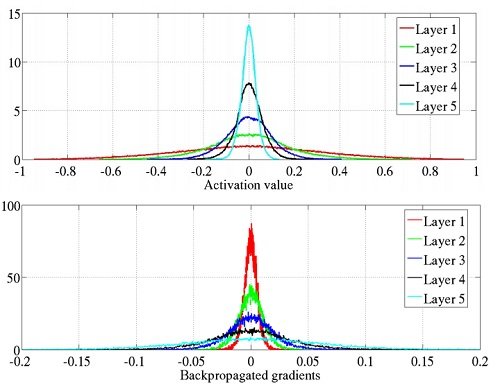• 梯度不为“零”：激活值的方差是逐层递减的，这导致反向传播中的梯度也逐层递减。要解决梯度消失，就要避免激活值方差的衰减，最理想的情况是，每层的输出值（激活值）保持高斯分布。梯度不为0，反推神经网络各层激活值不会出现饱和现象且激活值不为0

# 为什么权重零初始化不是一个好的初始化过程？

•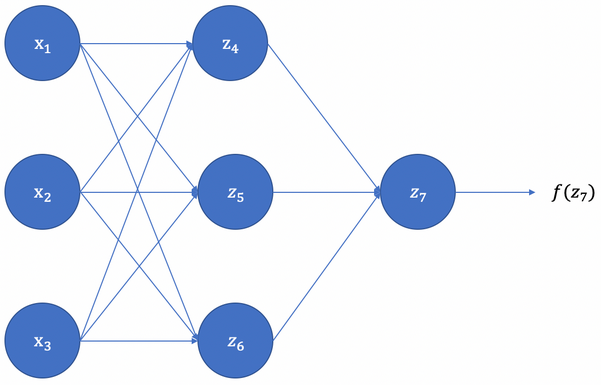• 前向计算

• z4、z5、z6计算

$\begin{array}{l} z_{4}=w_{14} * x_{1}+w_{24} * x_{2}+w_{34} * x_{3}+b_{4} \\ z_{5}=w_{15} * x_{1}+w_{25} * x_{2}+w_{35} * x_{3}+b_{5} \\ z_{6}=w_{16} * x_{1}+w_{26} * x_{2}+w_{36} * x_{3}+b_{6} \end{array}$

• 由于权重和偏置的初始值都为0，且同一层网络的激活函数相同，z4=z5=z6=0，也即激活值相等

$a_{4}=a_{5}=a_{6}$

• 神经网络的最终输出$a_7$

$\begin{array}{l} z_{7}=w_{47} * a_{4}+w_{57} * a_{5}+w_{67} * a_{6} \\ a_{7}=f\left(z_{7}\right) \end{array}$

• 后向传播

• 根据反向传播算法和链式法则，计算损失函数对$w_{47}、w_{57}、w_{67}$ 的梯度

$\begin{array}{l} \frac{\partial \operatorname{Loss}\left(a_{7}, y\right)}{\partial w_{47}} =\frac{\partial \operatorname{Loss}\left(a_{7}, y\right)}{\partial a_{7}} * \frac{\partial a_{7}}{\partial z_{7}} * \frac{\partial z_{7}}{\partial w_{47}} =\frac{\partial \operatorname{Loss}\left(a_{7}, y\right)}{\partial a_{7}} * \frac{\partial a_{7}}{\partial z_{7}} * a_{4} \\ \\ \frac{\partial \operatorname{Loss}\left(a_{7}, y\right)}{\partial w_{57}} =\frac{\partial \operatorname{Loss}\left(a_{7}, y\right)}{\partial a_{7}} * \frac{\partial a_{7}}{\partial z_{7}} * \frac{\partial z_{7}}{\partial w_{57}}=\frac{\partial \operatorname{Loss}\left(a_{7}, y\right)}{\partial a_{7}} * \frac{\partial a_{7}}{\partial z_{7}} * a_{5}\\ \\ \frac{\partial \operatorname{Loss}\left(a_{7}, y\right)}{\partial w_{67}}=\frac{\partial \operatorname{Loss}\left(a_{7}, y\right)}{\partial a_{7}} * \frac{\partial a_{7}}{\partial z_{7}} * a_{6} \end{array}$

• 由于$a_{4}=a_{5}=a_{6}$